# NCERT Exemplar Class 11 Physics Solutions for Chapter 6 - Work Energy And Power

NCERT Exemplar Solutions for Class 11 Physics Chapter 6 Work, Energy and Power is a prime study material that is essential for students who are preparing for CBSE Class 11 annual examination as well as Engineering/Medical entrance examinations. Solving these exemplar questions will help you understand the topic thoroughly. This NCERT Exemplar Class 11 Physics Chapter 6 provides you with exemplar questions, numerical problems, MCQs, worksheets, HOTS and short and long answer questions that will help you study the topic with respect to the annual examination and to prepare notes. Work, Power and Energy are all important concepts in Physics, and they all have a relationship with each other. Work can simply be defined as the product of force and displacement, and when the force and displacement occur in the same direction, work done is positive. Power can simply be defined as the rate at which work is done, while Energy is the ability of the body to do work. Download the NCERT Exemplar Class 11 Chapter 6 Work, Power and Energy PDF, which is available below:

## Download the PDF of NCERT Exemplar Class 11 Physics Chapter 6 Work, Energy and Power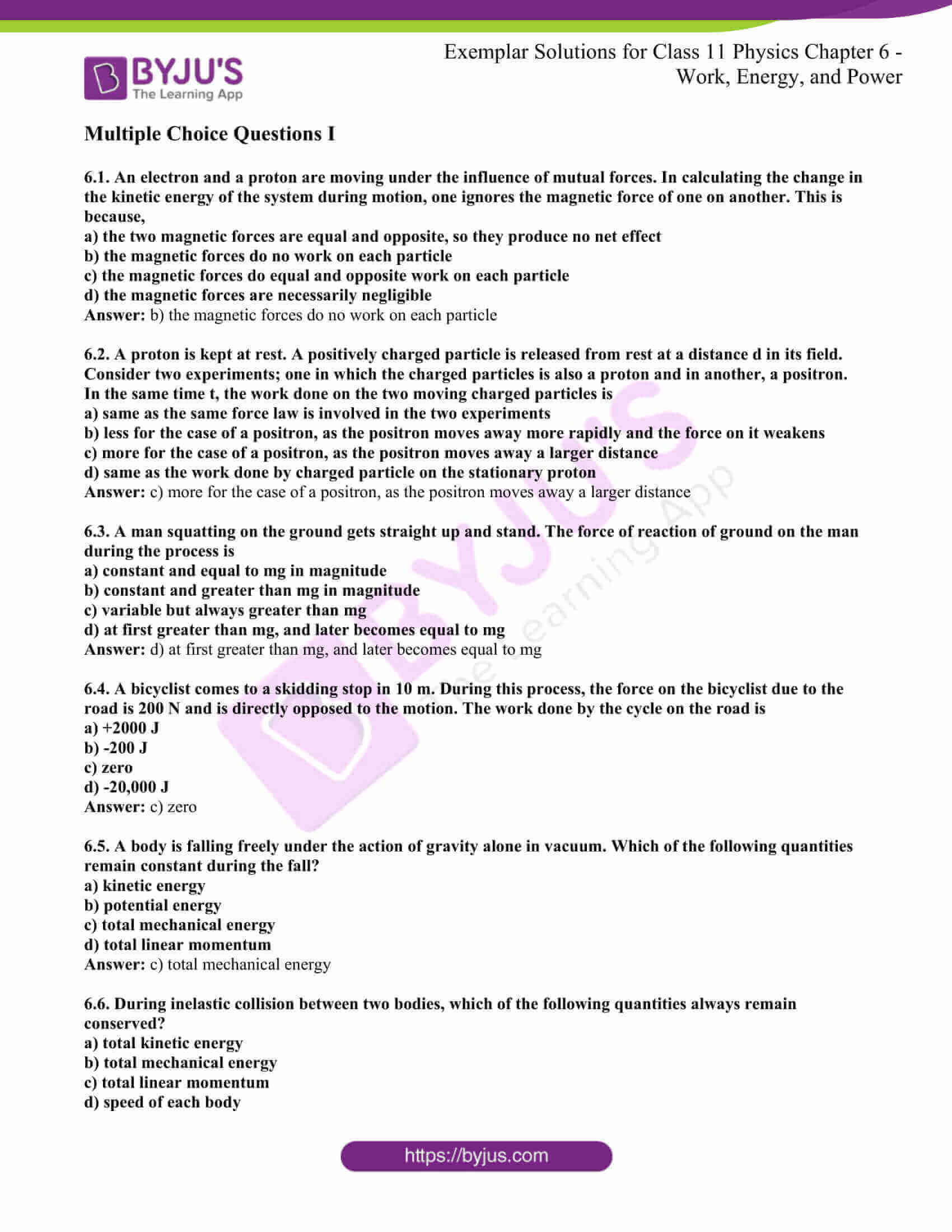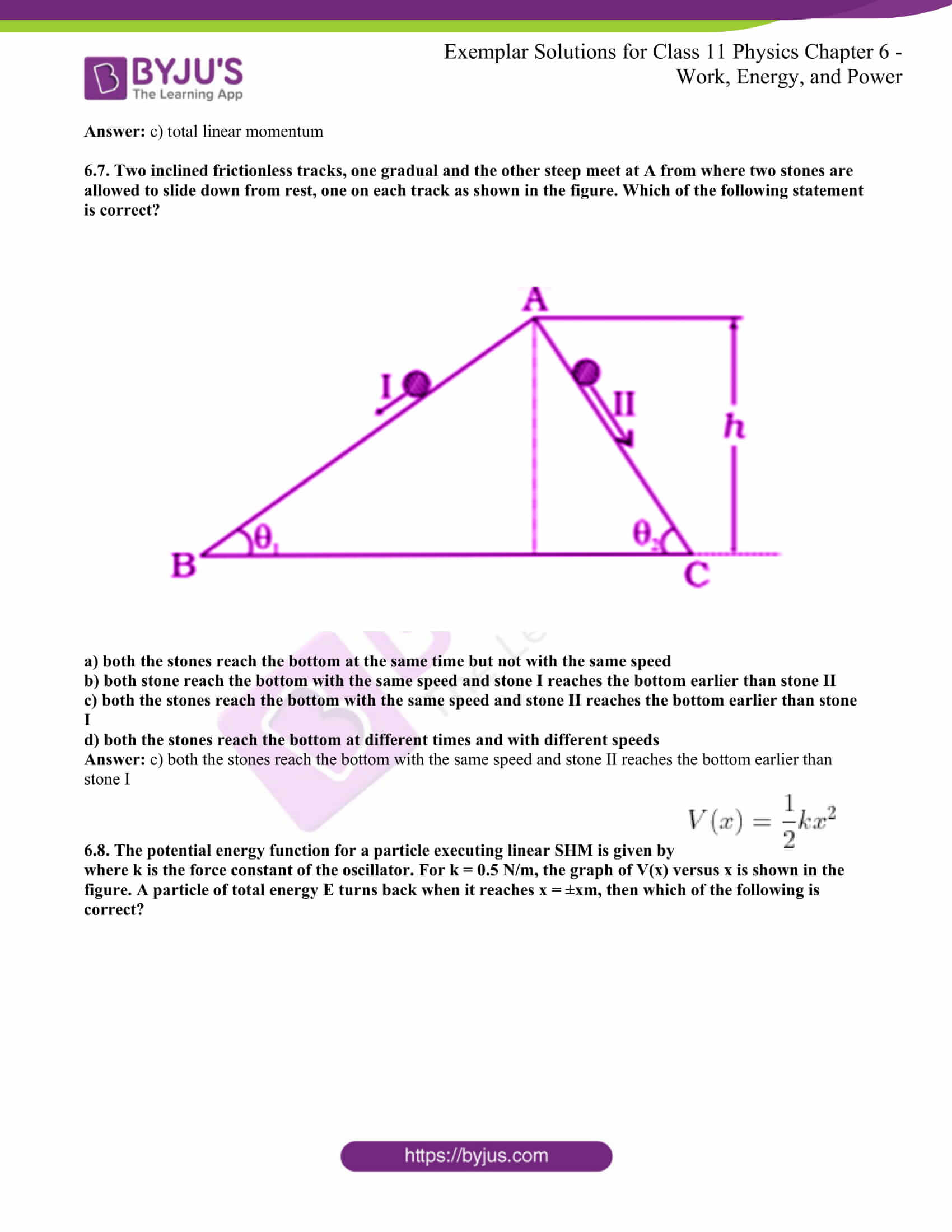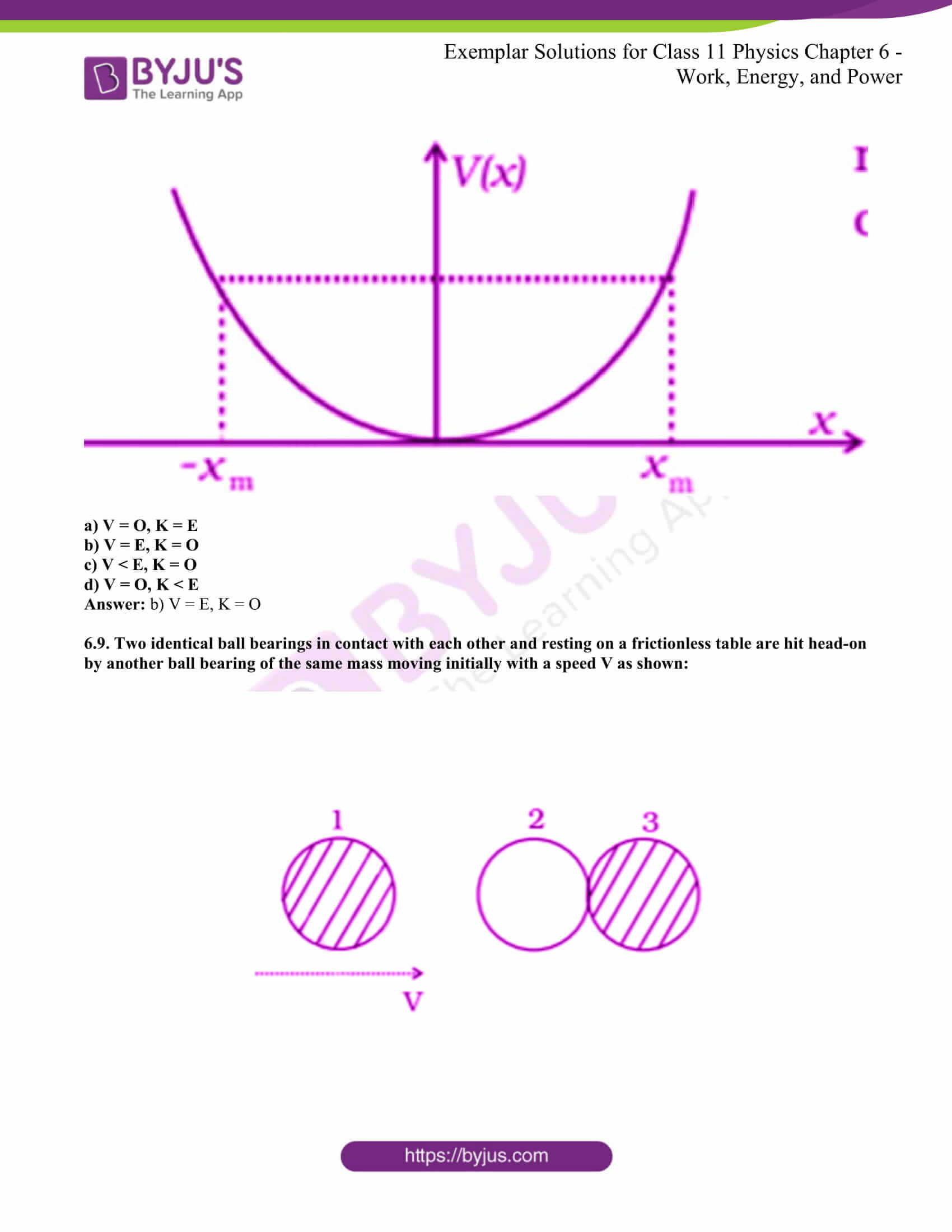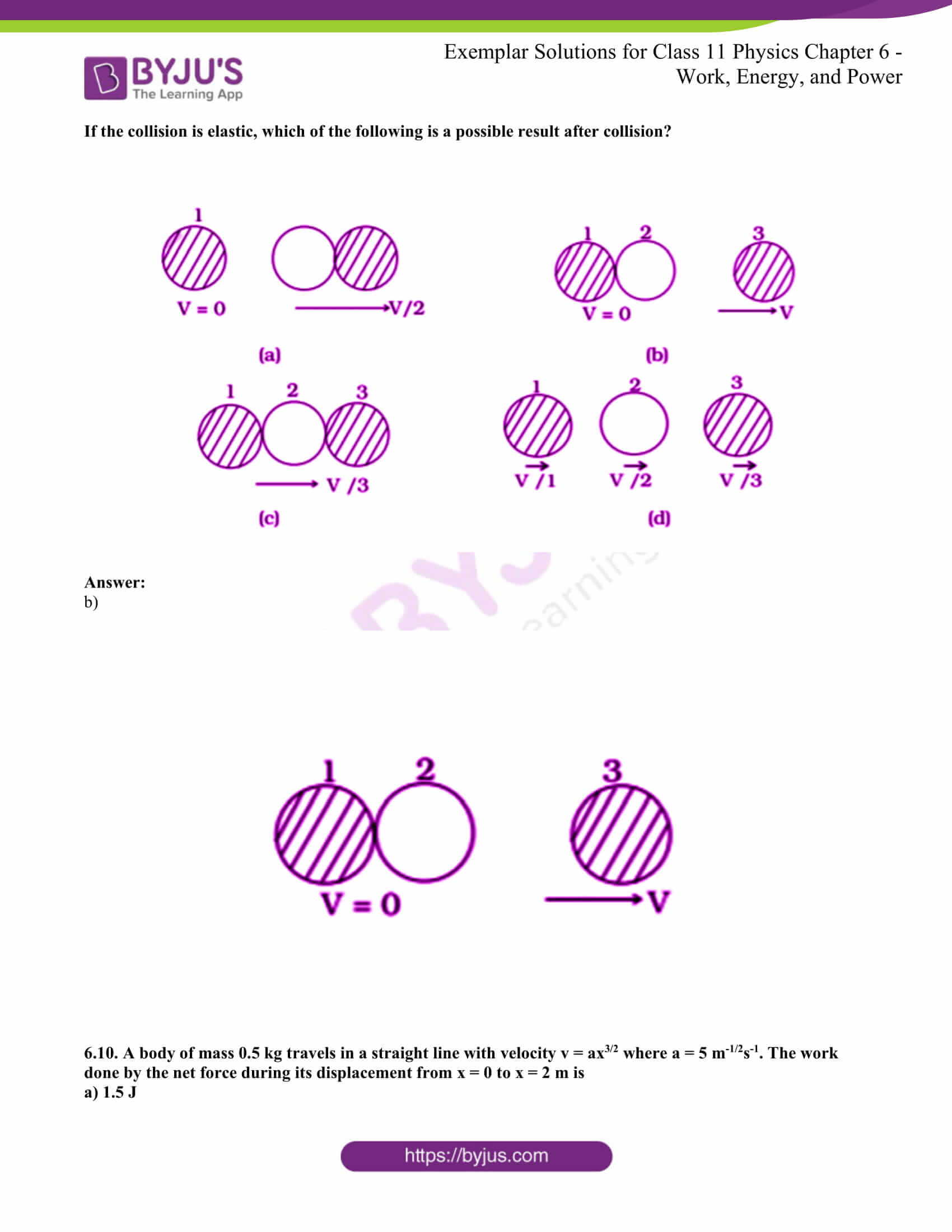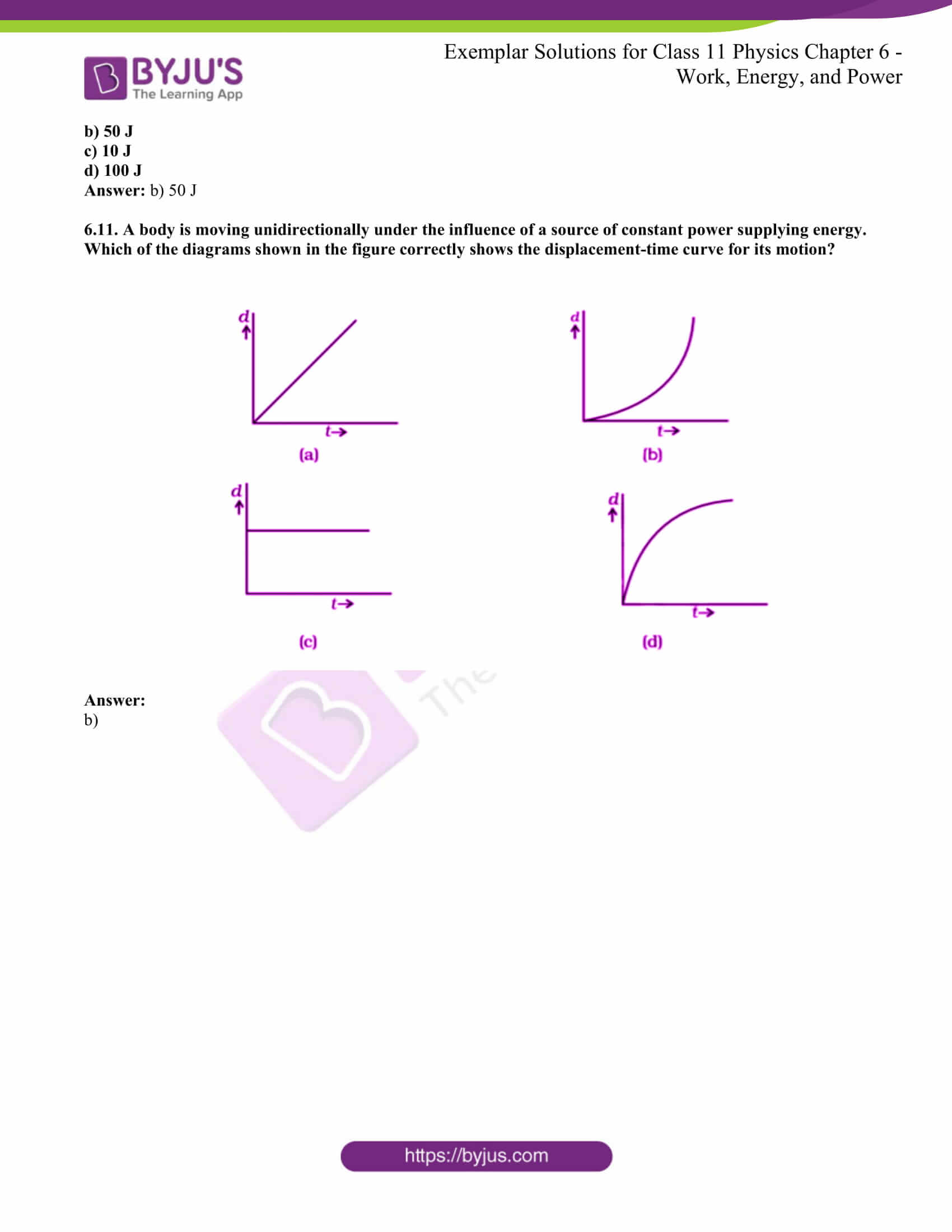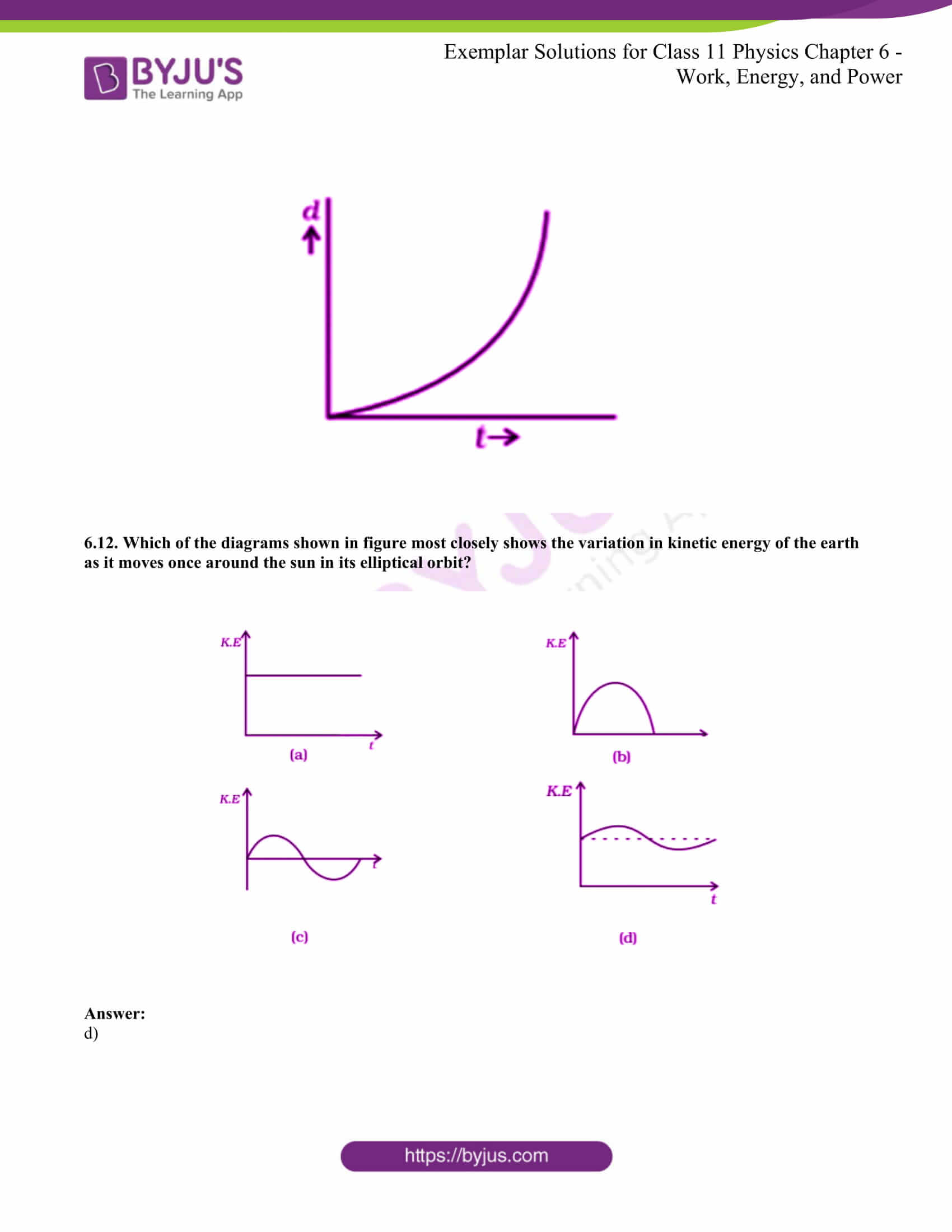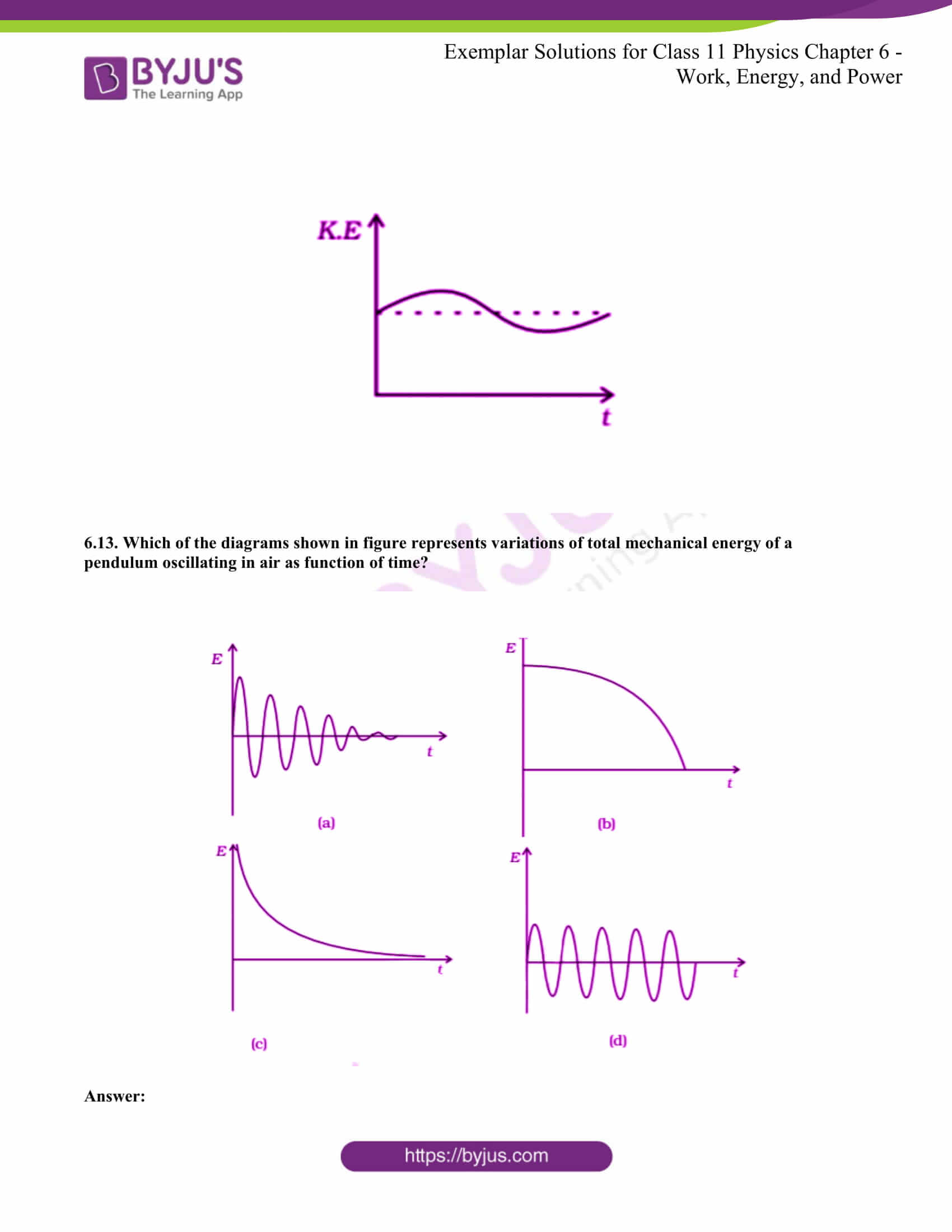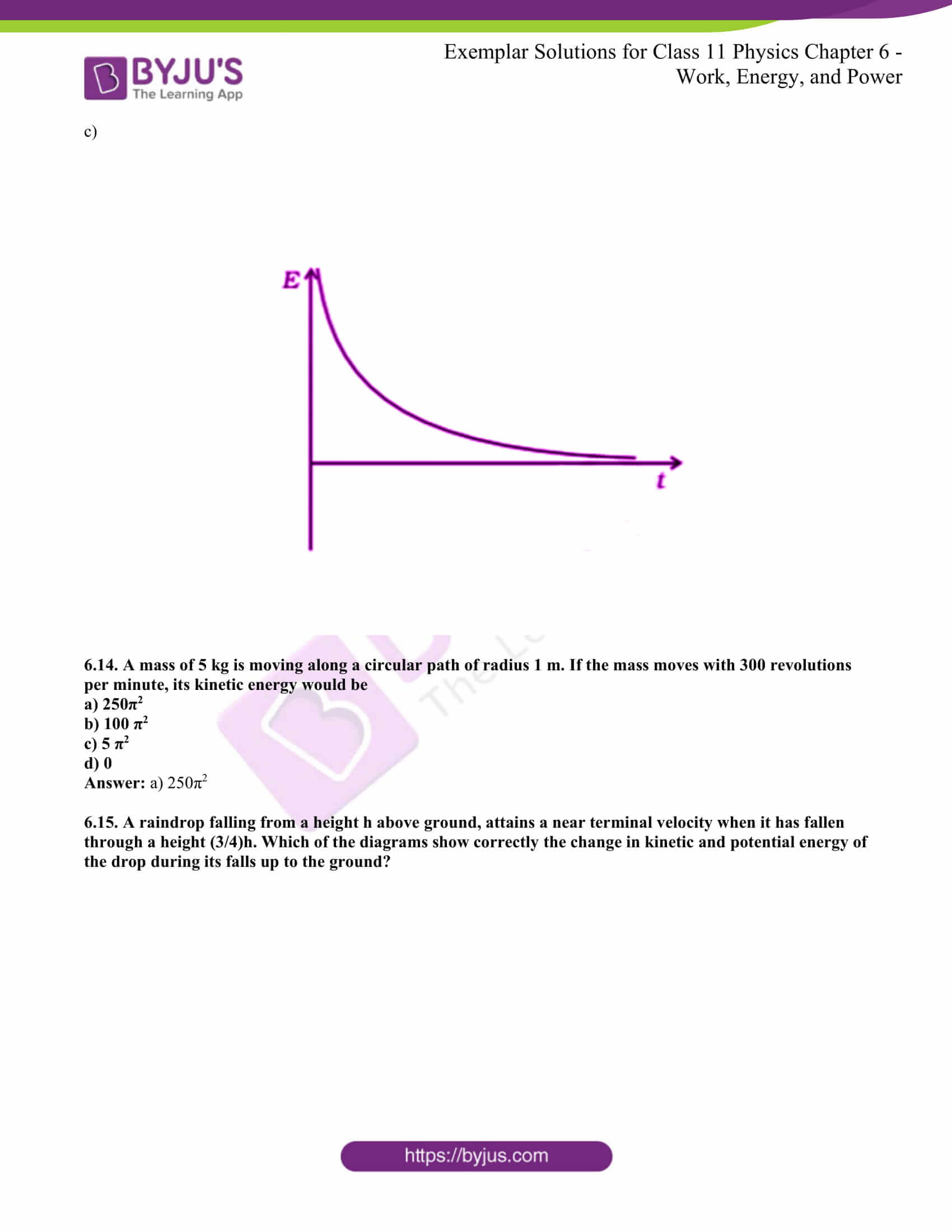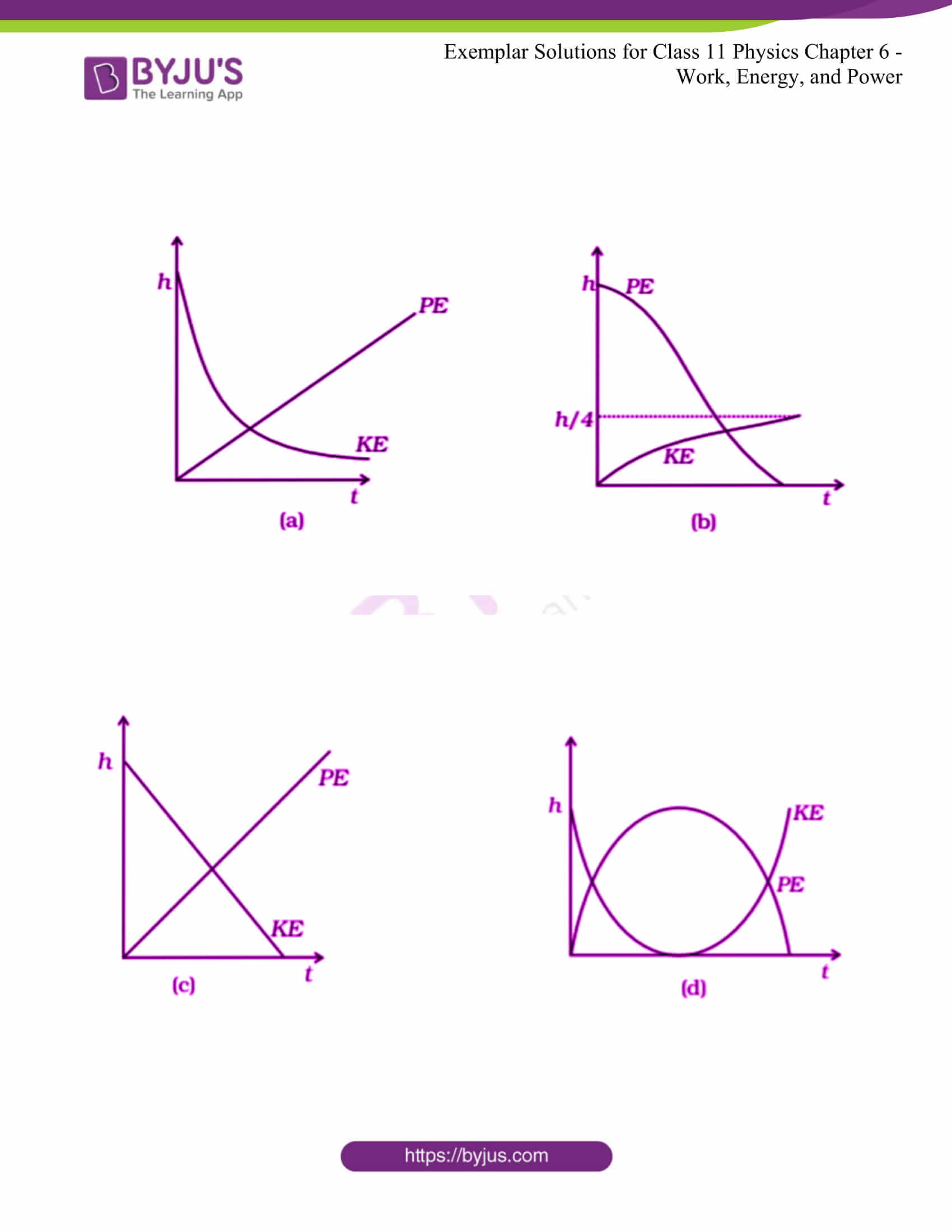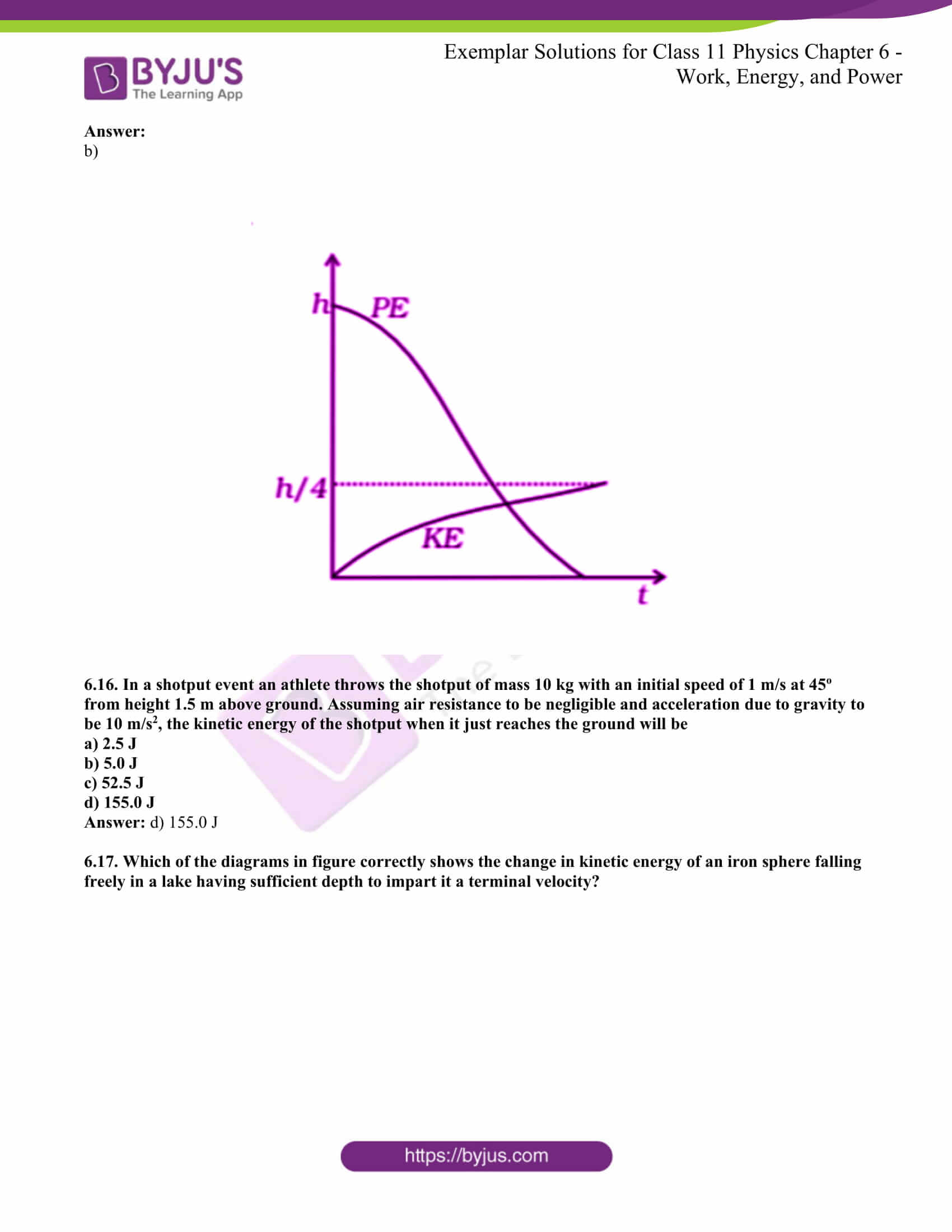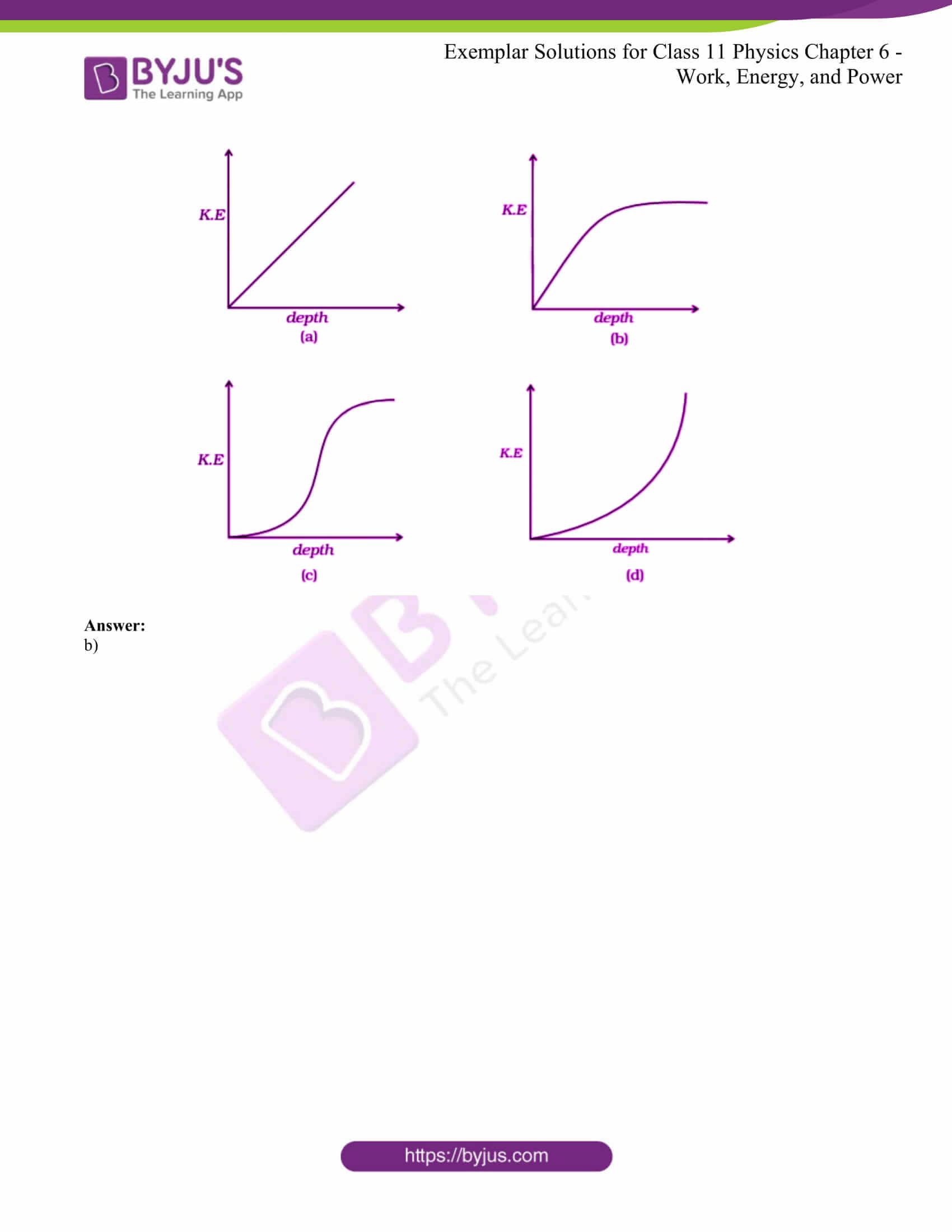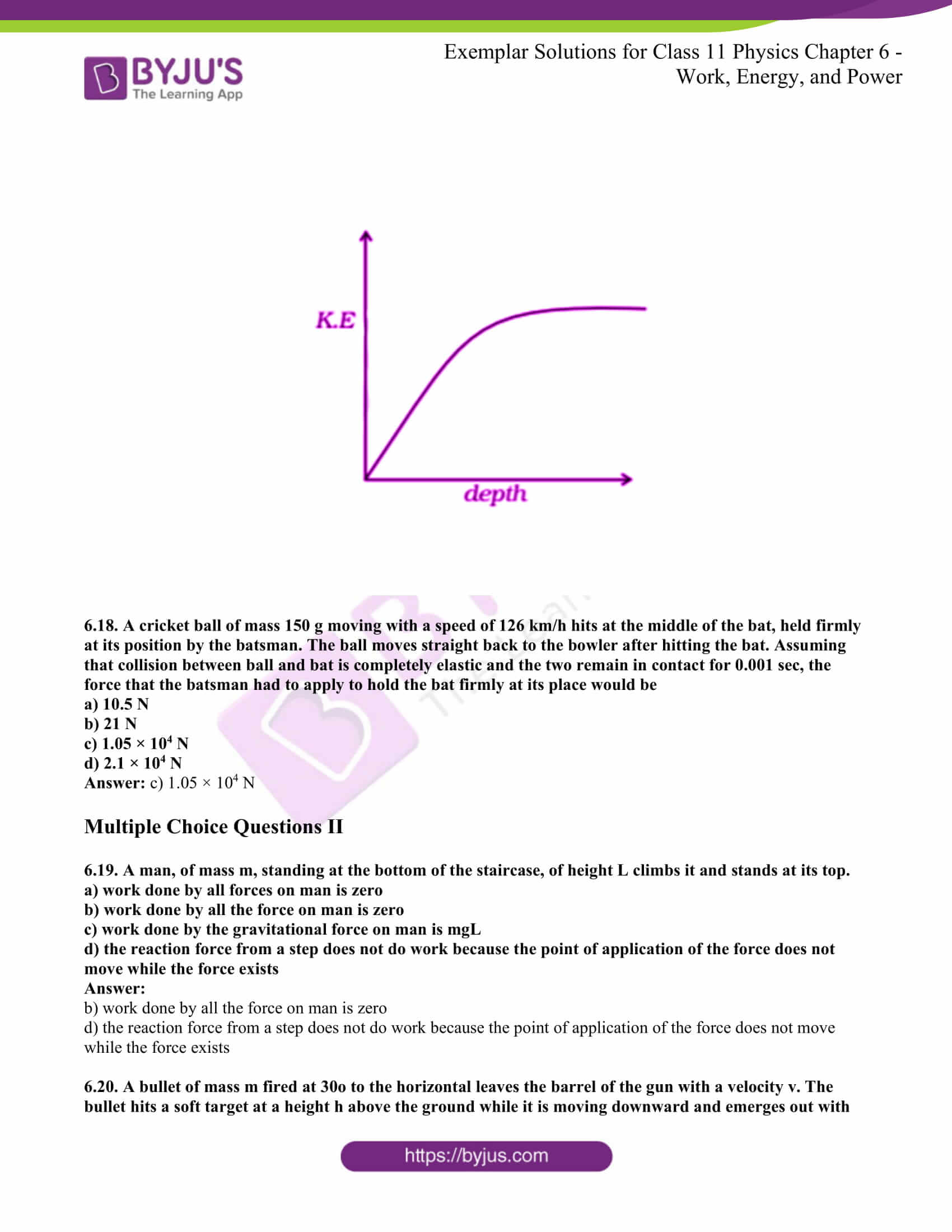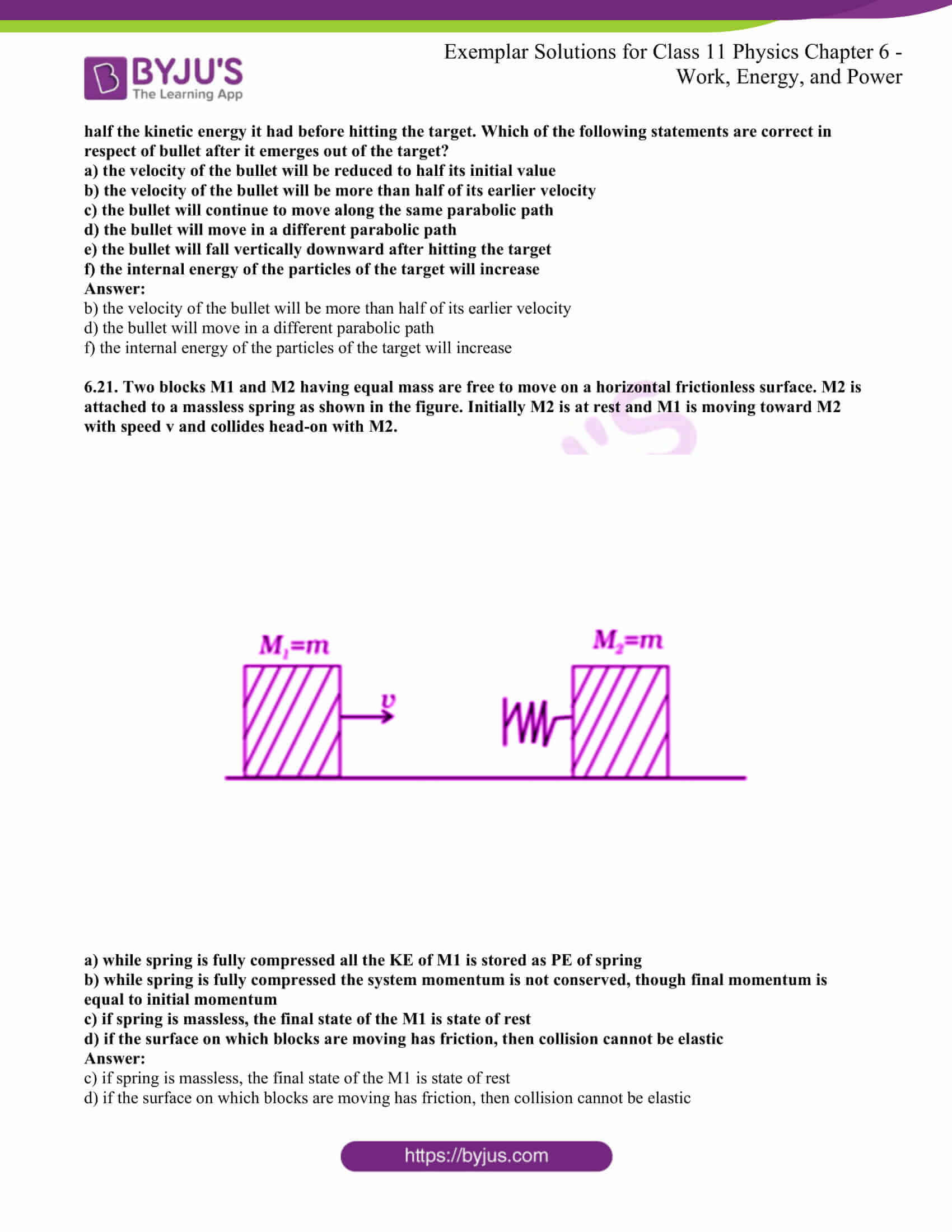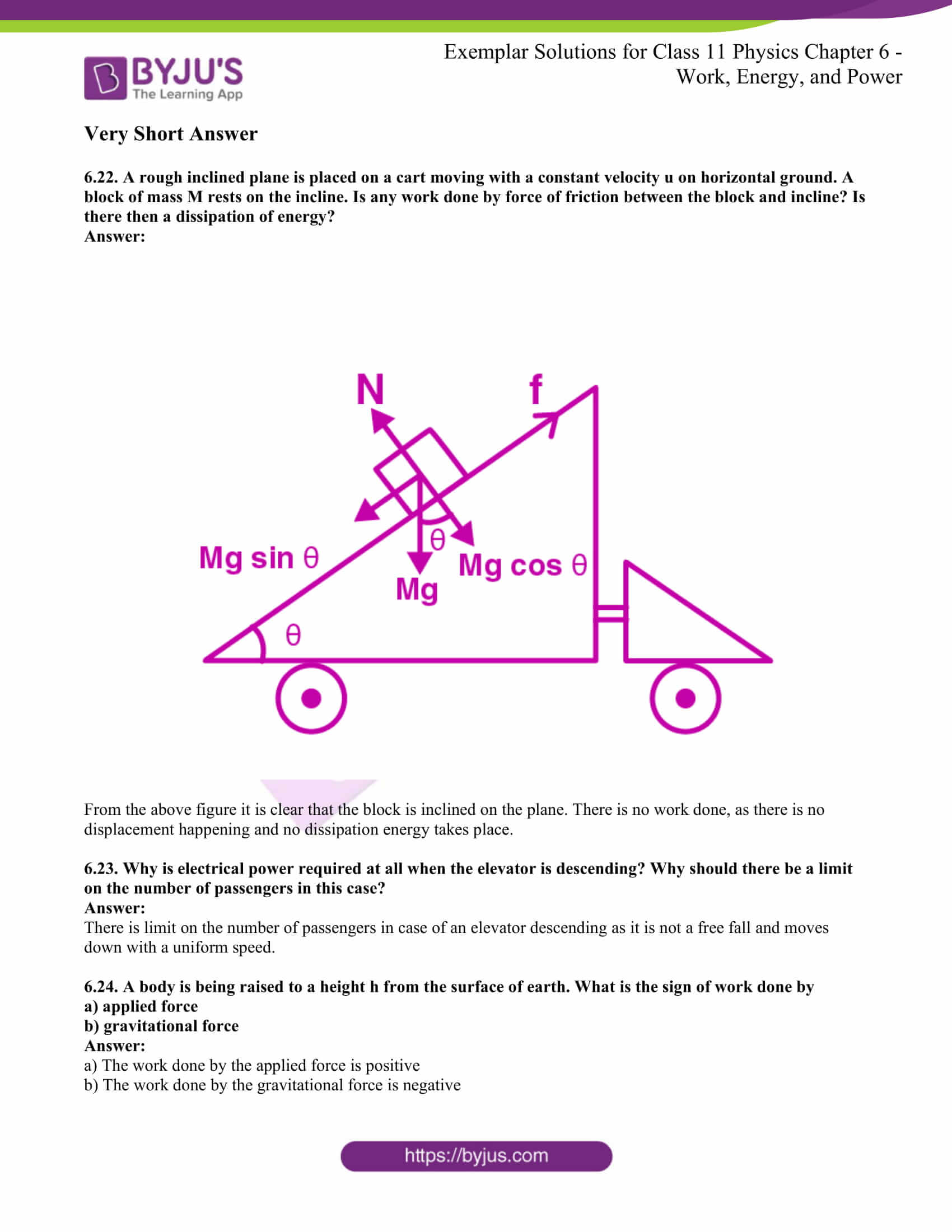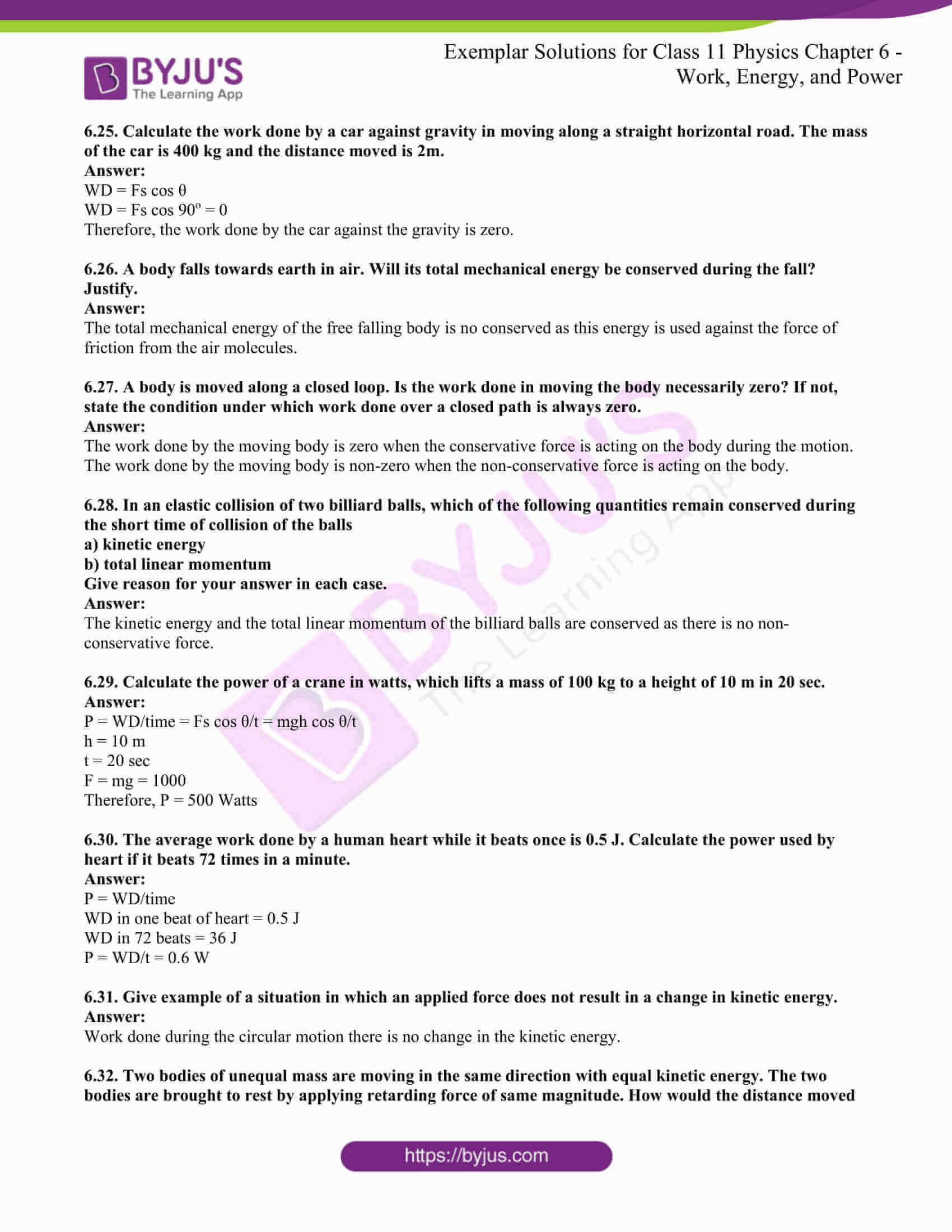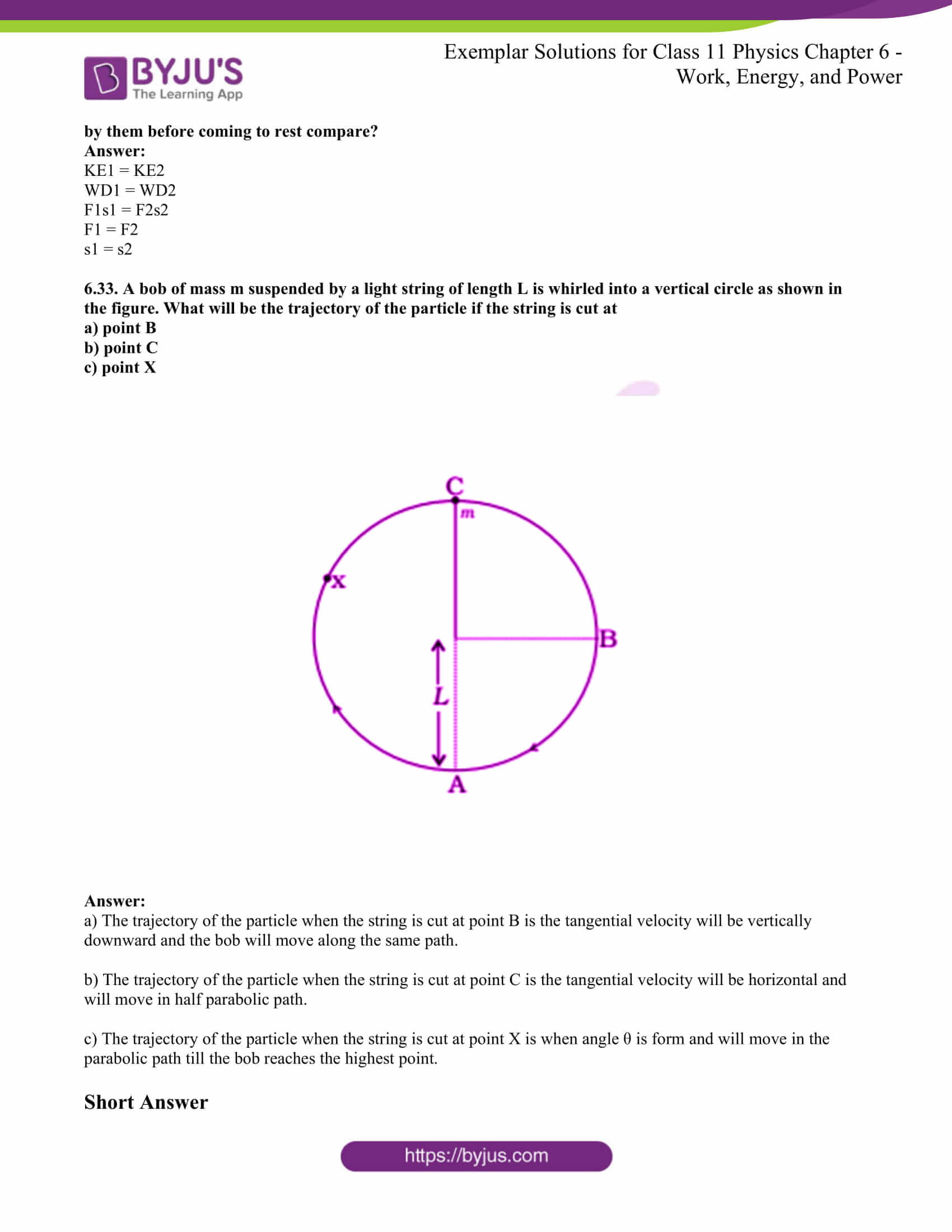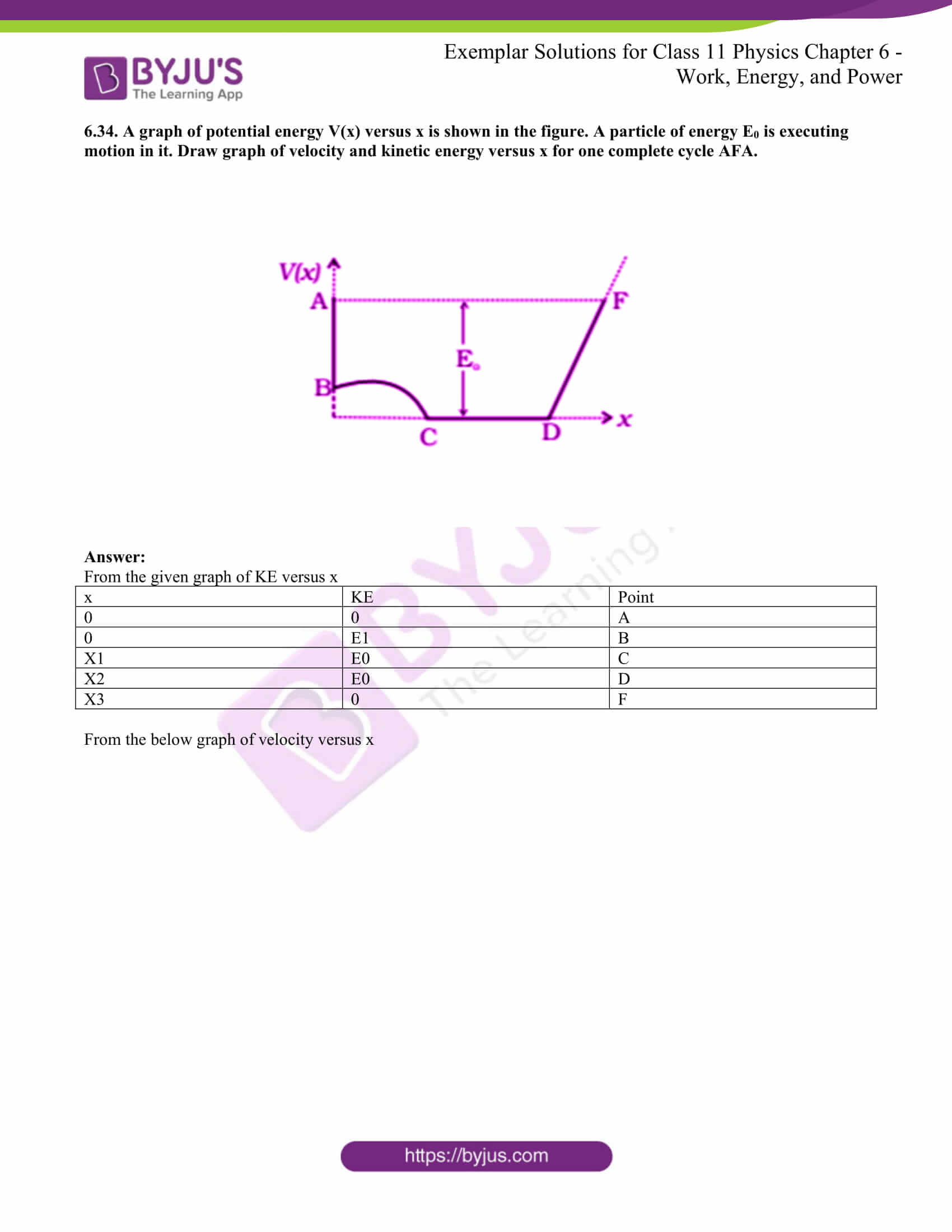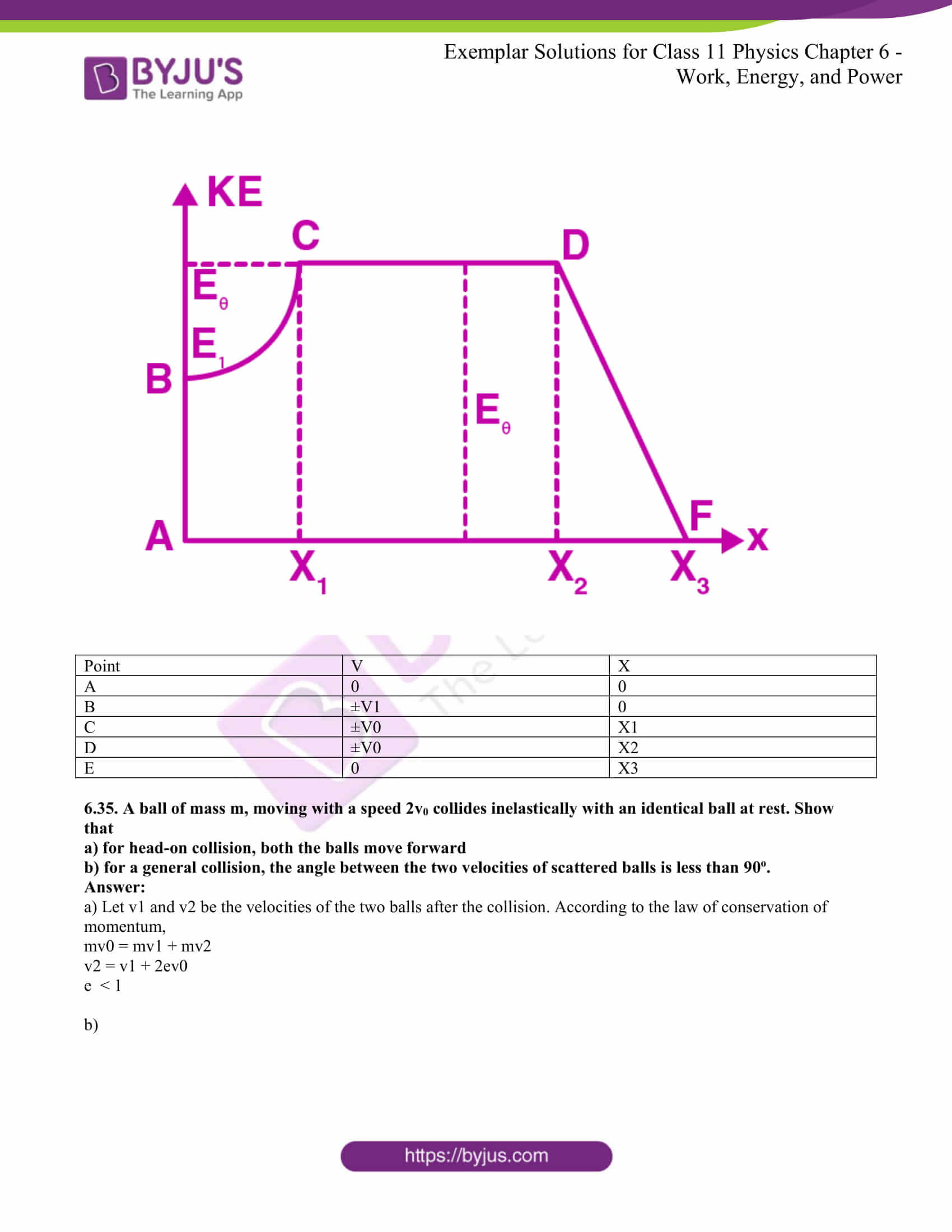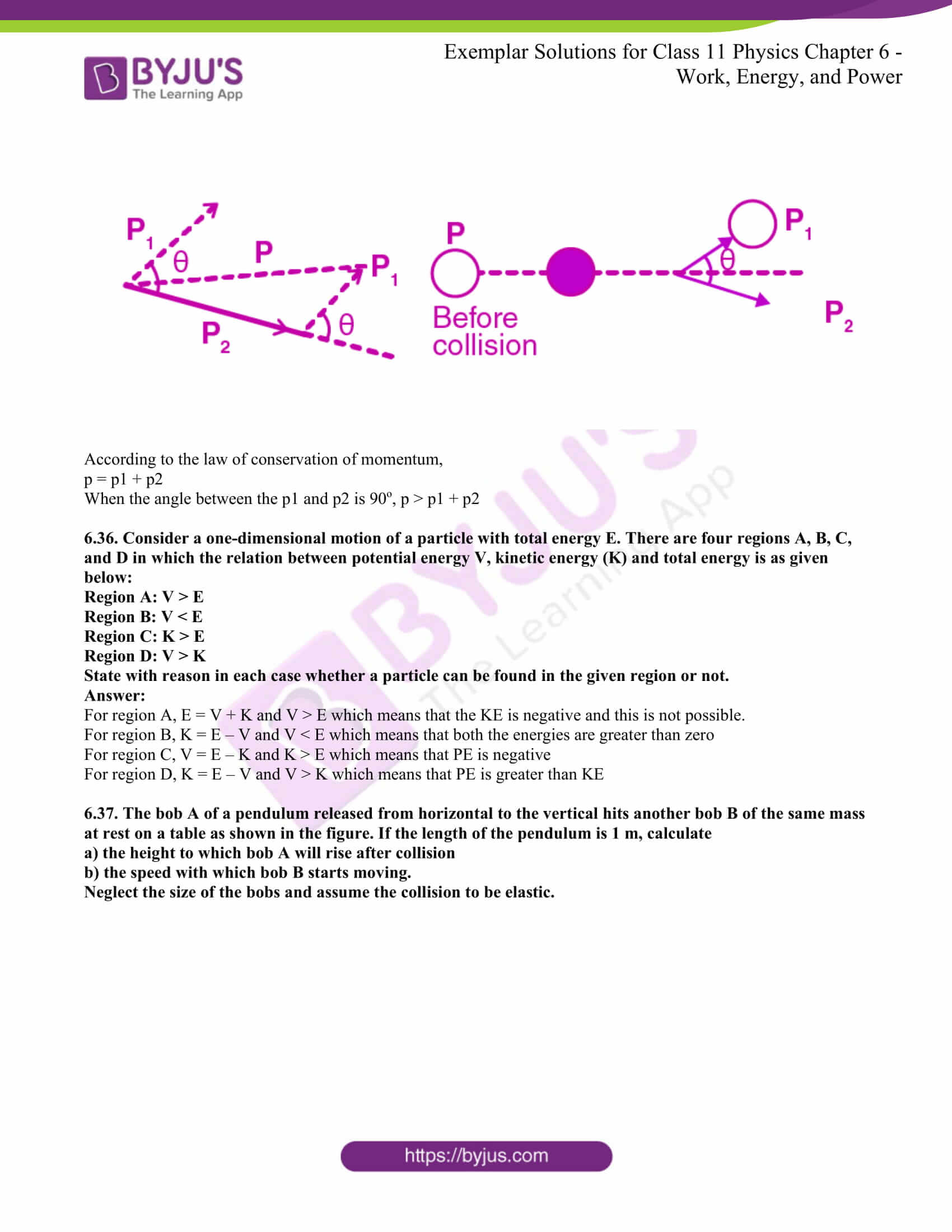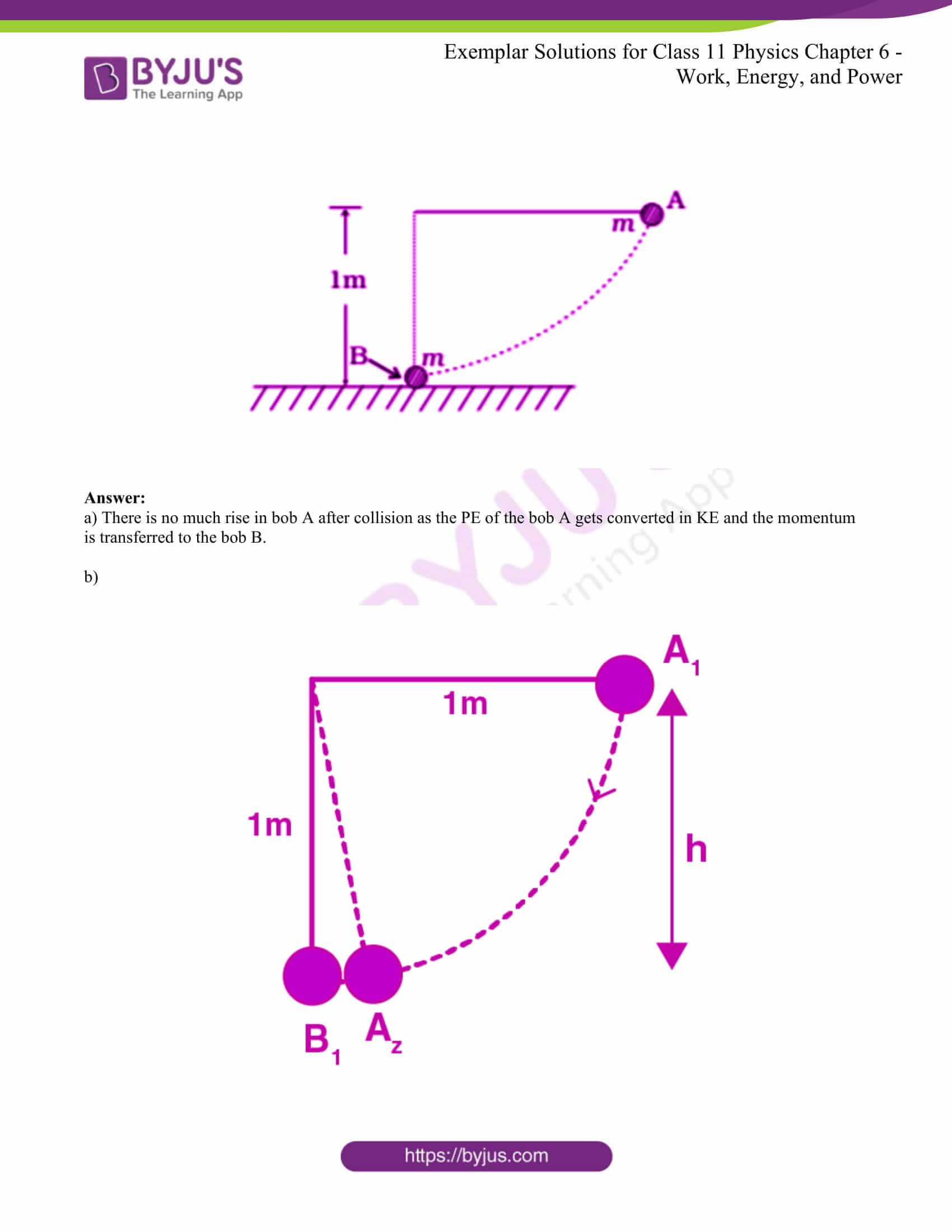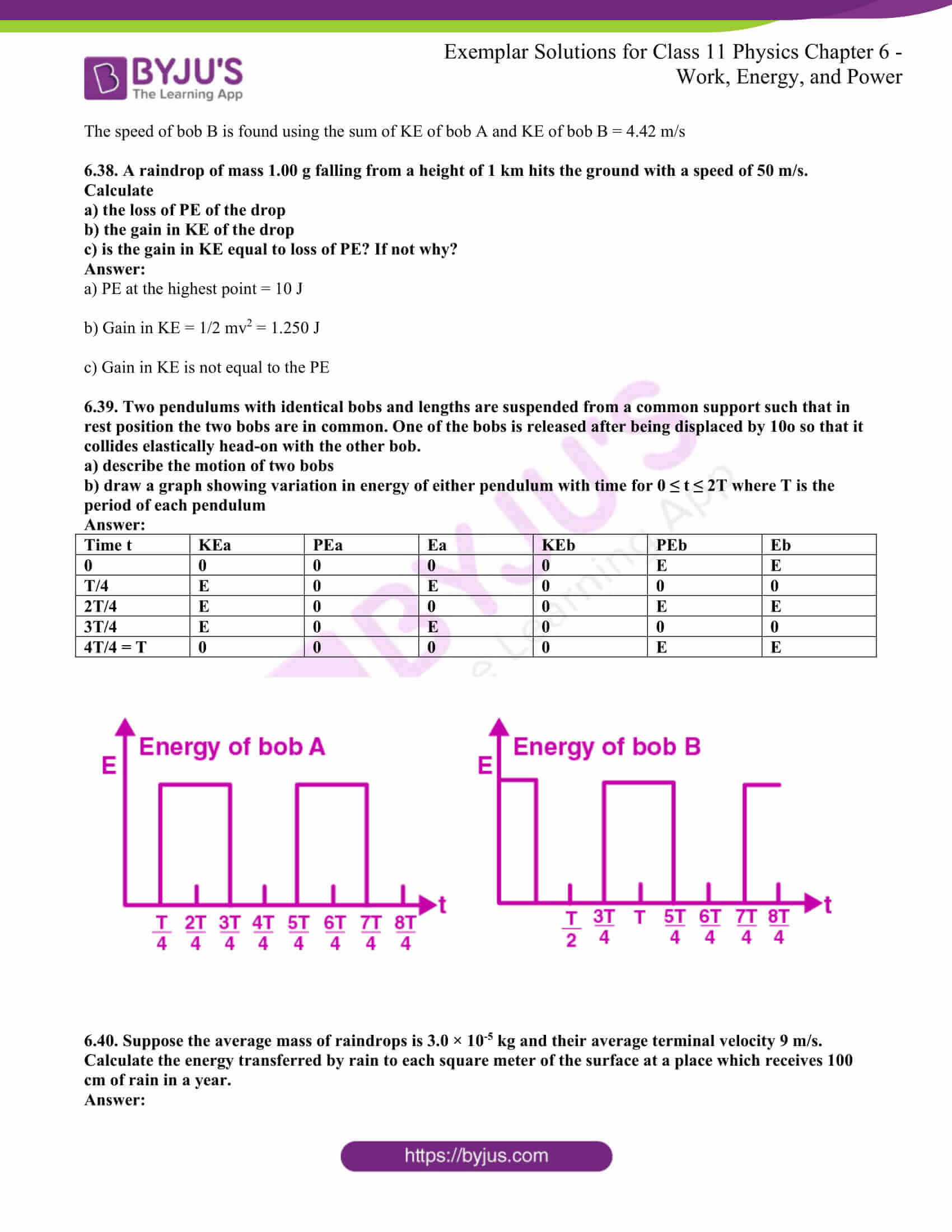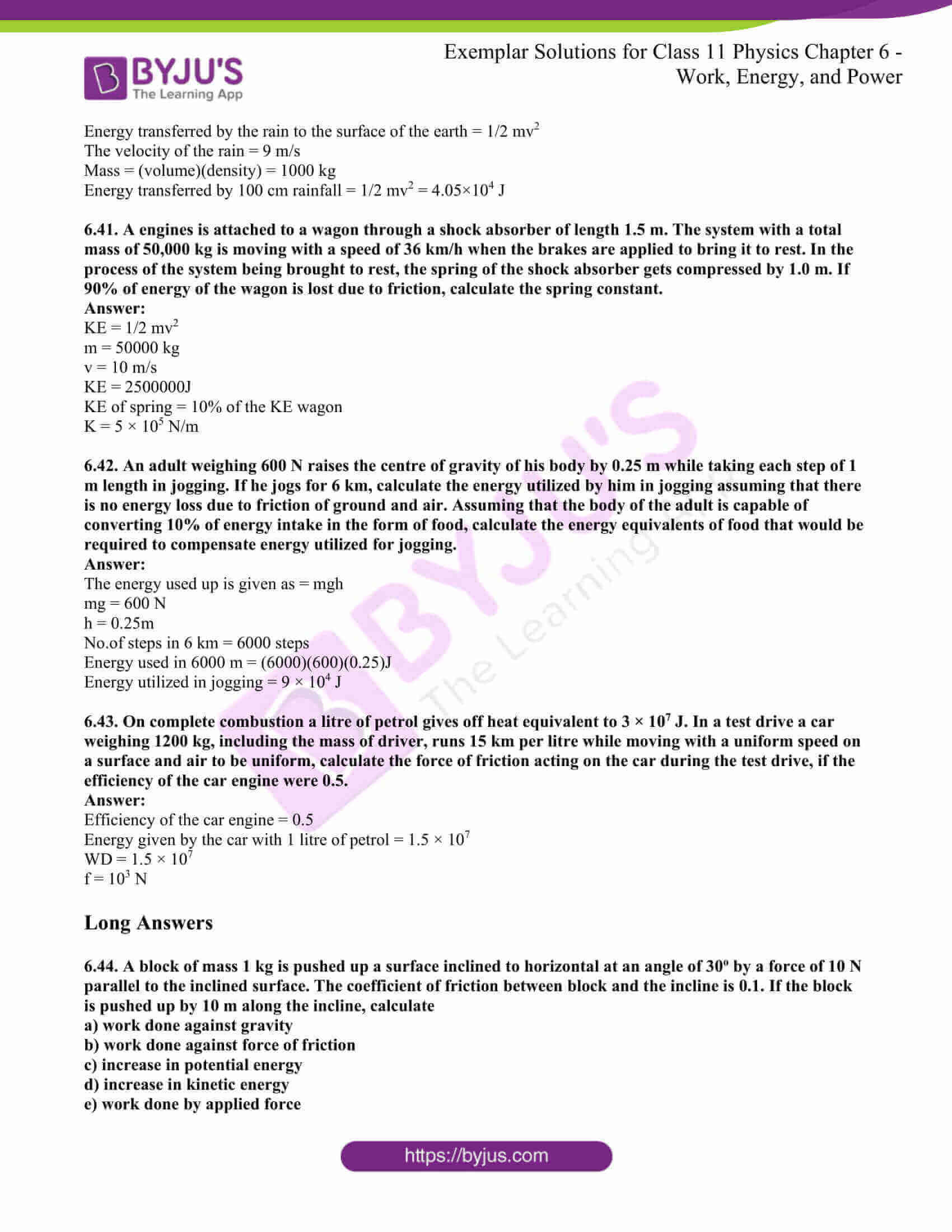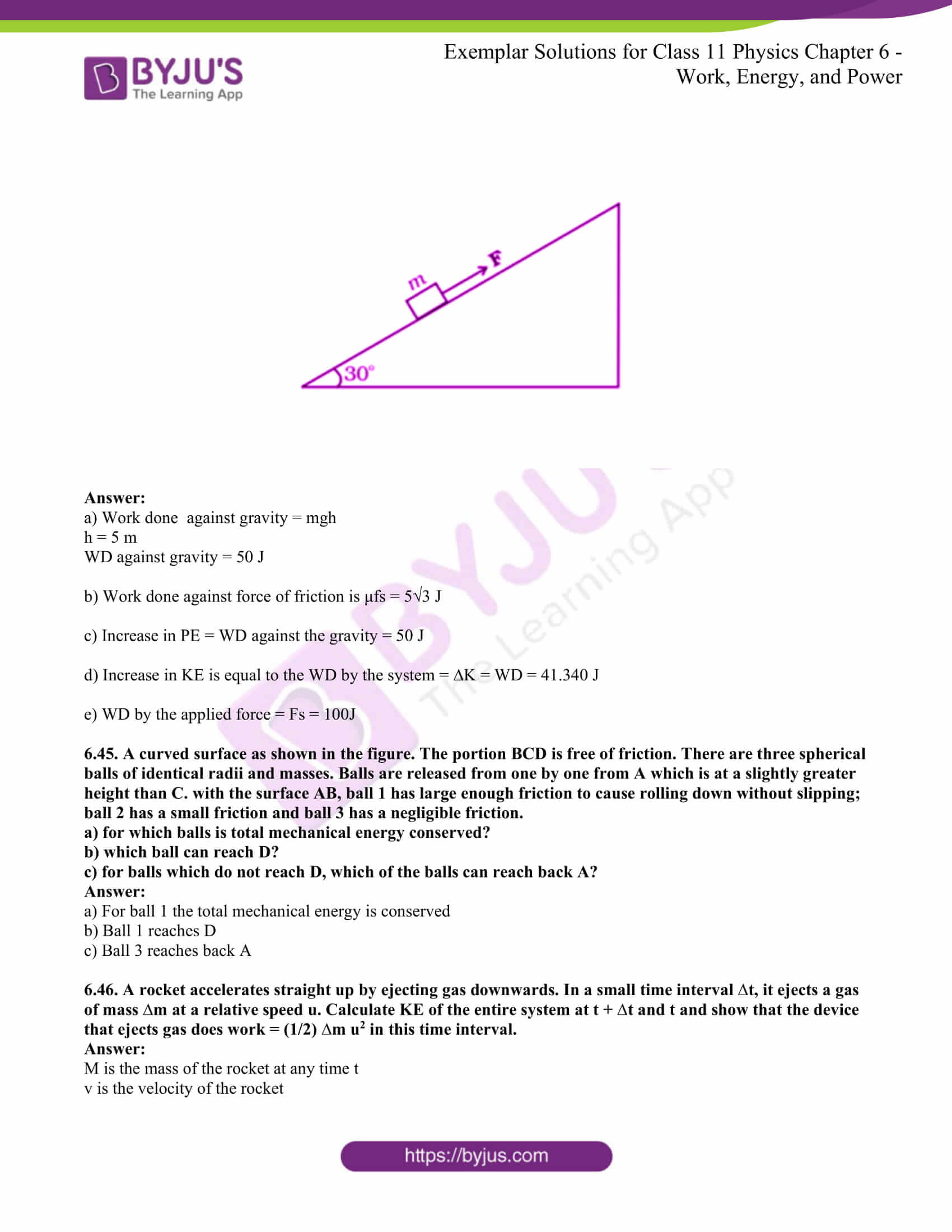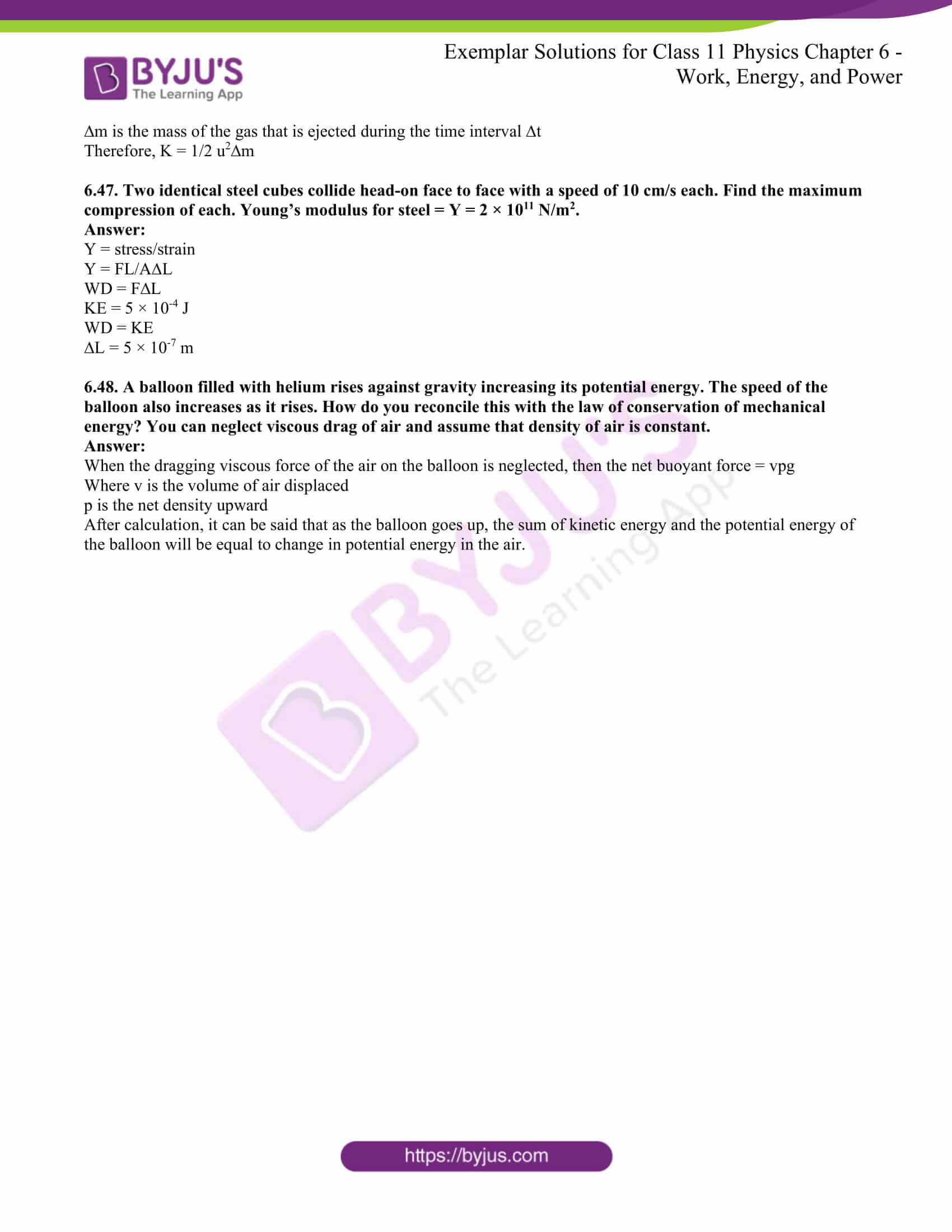### Multiple-choice Questions I

6.1. An electron and a proton are moving under the influence of mutual forces. In calculating the change in the kinetic energy of the system during motion, one ignores the magnetic force of one on another. This is because,

a) the two magnetic forces are equal and opposite, so they produce no net effect

b) the magnetic forces do no work on each particle

c) the magnetic forces do equal and opposite work on each particle

d) the magnetic forces are necessarily negligible

Answer: b) the magnetic forces do no work on each particle

6.2. A proton is kept at rest. A positively charged particle is released from rest at a distance d in its field. Consider two experiments; one in which the charged particles is also a proton and in another, a positron. In the same time t, the work done on the two moving charged particles is

a) the same as the same force law is involved in the two experiments

b) less for the case of a positron, as the positron moves away more rapidly and the force on it weakens

c) more for the case of a positron, as the positron moves away from a larger distance

d) same as the work done by charged particle on the stationary proton

Answer: c) more for the case of a positron, as the positron moves away from a larger distance

6.3. A man squatting on the ground gets straight up and stand. The force of reaction of ground on the man during the process is

a) constant and equal to mg in magnitude

b) constant and greater than mg in magnitude

c) variable but always greater than mg

d) at first greater than mg, and later becomes equal to mg

Answer: d) at first greater than mg, and later becomes equal to mg

6.4. A bicyclist comes to a skidding stop in 10 m. During this process, the force on the bicyclist due to the road is 200 N and is directly opposed to the motion. The work done by the cycle on the road is

a) +2000 J

b) -200 J

c) zero

d) -20,000 J

6.5. A body is falling freely under the action of gravity alone in a vacuum. Which of the following quantities remain constant during the fall?

a) kinetic energy

b) potential energy

c) total mechanical energy

d) total linear momentum

6.6. During inelastic collision between two bodies, which of the following quantities always remain conserved?

a) total kinetic energy

b) total mechanical energy

c) total linear momentum

d) speed of each body

6.7. Two inclined frictionless tracks, one gradual and the other steep, meet at A, from where two stones are allowed to slide down from rest, one on each track as shown in the figure. Which of the following statement is correct?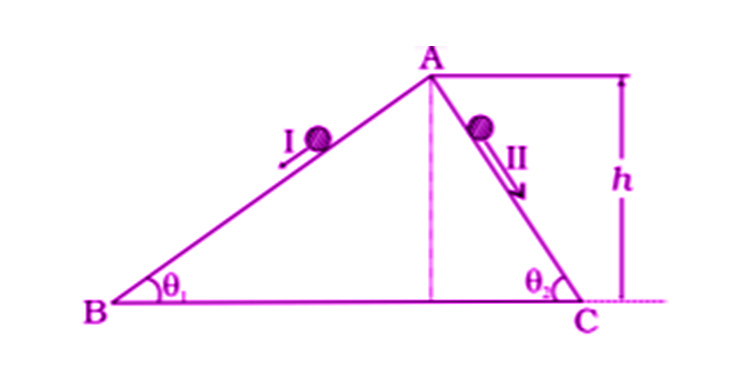a) both the stones reach the bottom at the same time but not with the same speed

b) both stones reach the bottom with the same speed and stone I reaches the bottom earlier than stone II

c) both the stones reach the bottom with the same speed and stone II reaches the bottom earlier than stone I

d) both the stones reach the bottom at different times and with different speeds

Answer: c) both the stones reach the bottom with the same speed and stone II reaches the bottom earlier than stone I

6.8. The potential energy function for a particle executing linear SHM is given by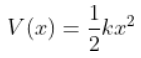where k is the force constant of the oscillator. For k = 0.5 N/m, the graph of V(x) versus x is shown in the figure. A particle of total energy E turns back when it reaches x = ±xm, then which of the following is correct?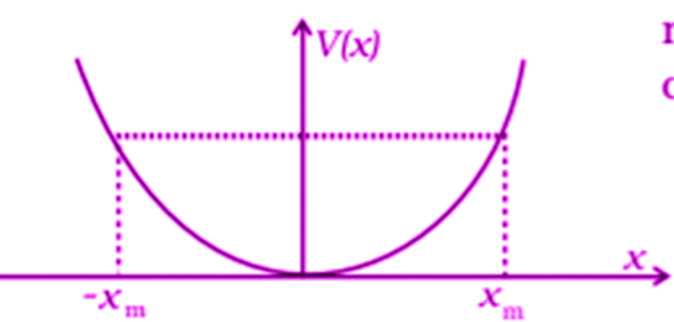a) V = O, K = E

b) V = E, K = O

c) V < E, K = O

d) V = O, K < E

Answer: b) V = E, K = O

6.9. Two identical ball bearings in contact with each other and resting on a frictionless table are hit head-on by another ball bearing of the same mass moving initially with a speed V as shown: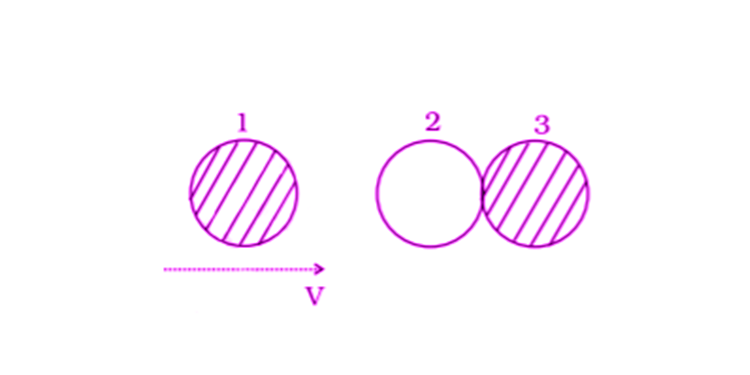If the collision is elastic, which of the following is a possible result after collision?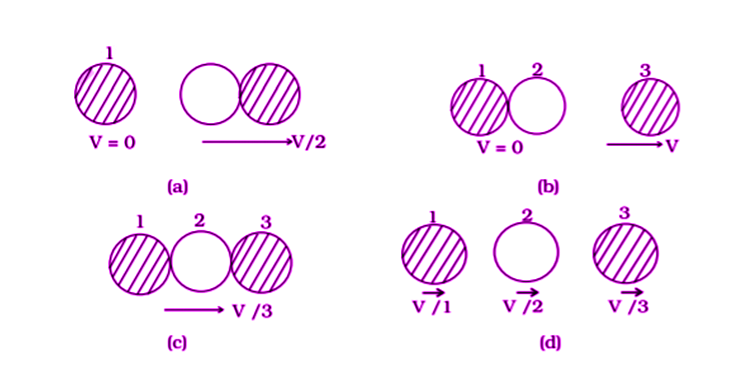b)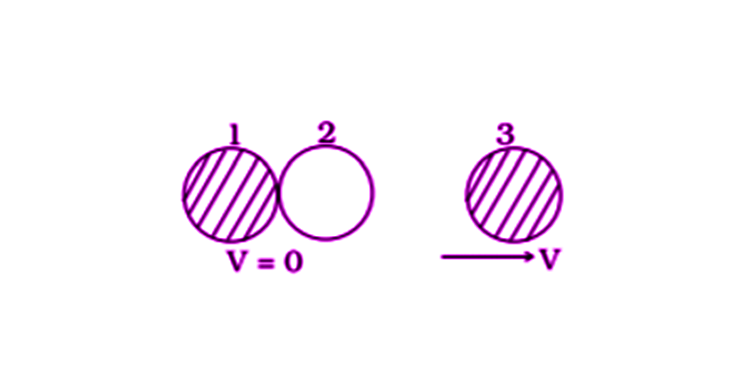6.10. A body of mass 0.5 kg travels in a straight line with velocity v = ax3/2 where a = 5 m-1/2s-1. The work done by the net force during its displacement from x = 0 to x = 2 m is

a) 1.5 J

b) 50 J

c) 10 J

d) 100 J

6.11. A body is moving unidirectionally under the influence of a source of constant power supplying energy. Which of the diagrams shown in the figure correctly shows the displacement-time curve for its motion?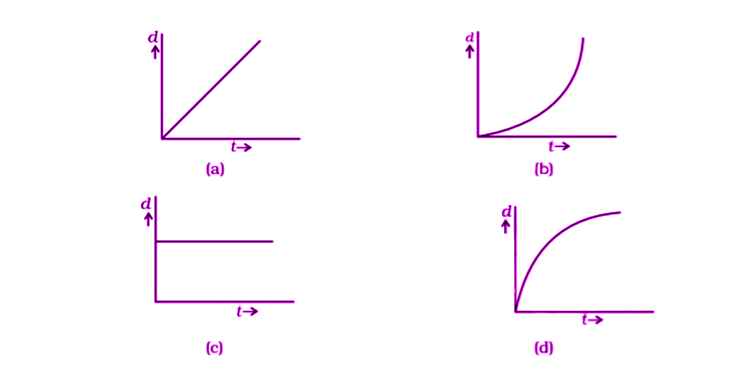b)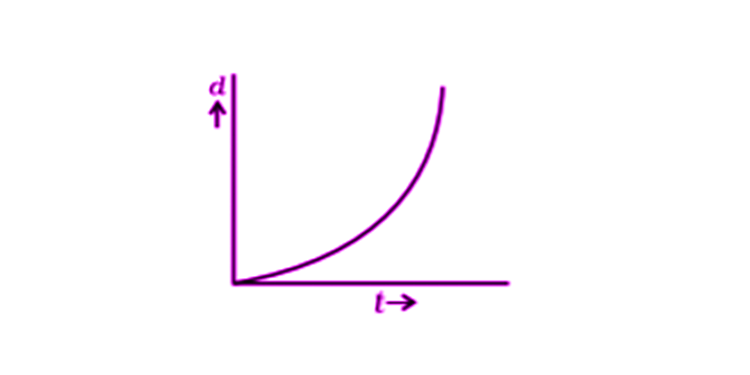6.12. Which of the diagrams shown in figure most closely shows the variation in kinetic energy of the earth as it moves once around the sun in its elliptical orbit?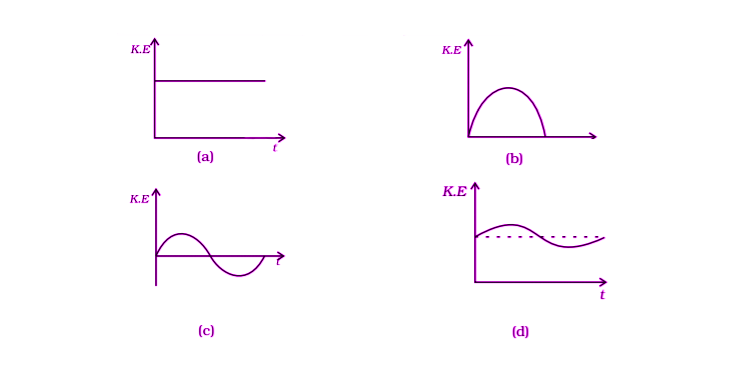d)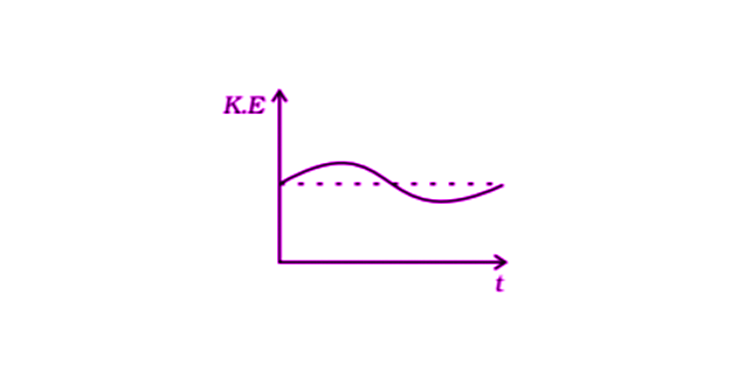6.13. Which of the diagrams shown in the figure represents variations of the total mechanical energy of a pendulum oscillating in air as a function of time?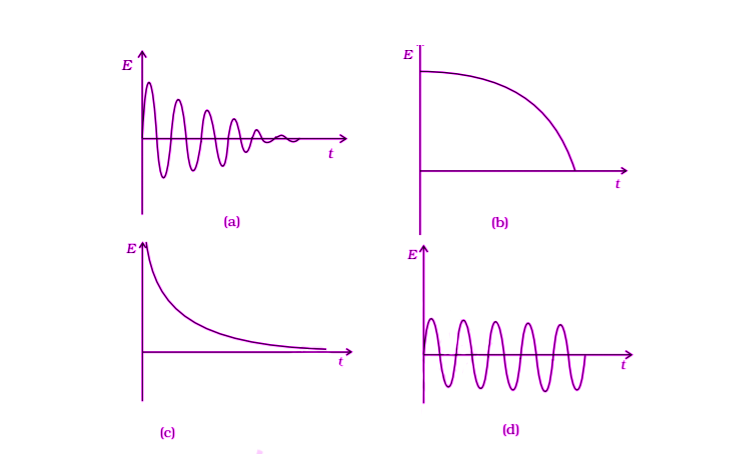c)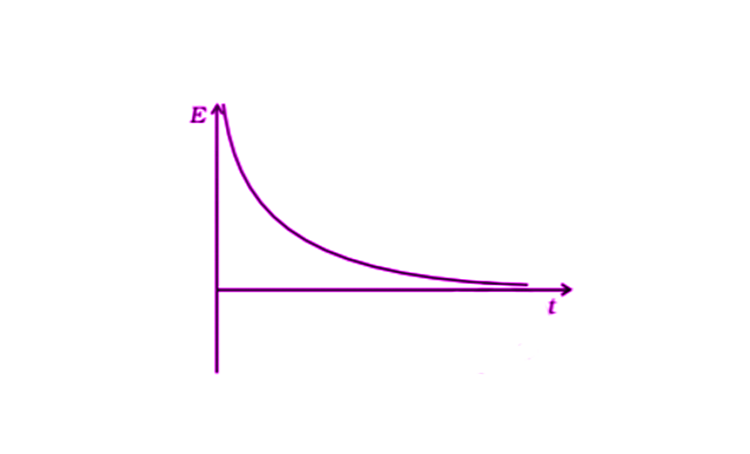6.14. A mass of 5 kg is moving along a circular path of radius 1 m. If the mass moves with 300 revolutions per minute, its kinetic energy would be

a) 250π2

b) 100 π2

c) 5 π2

d) 0

6.15. A raindrop falling from a height h above ground, attains a near-terminal velocity when it has fallen through a height (3/4)h. Which of the diagrams show correctly the change in kinetic and potential energy of the drop during its falls up to the ground?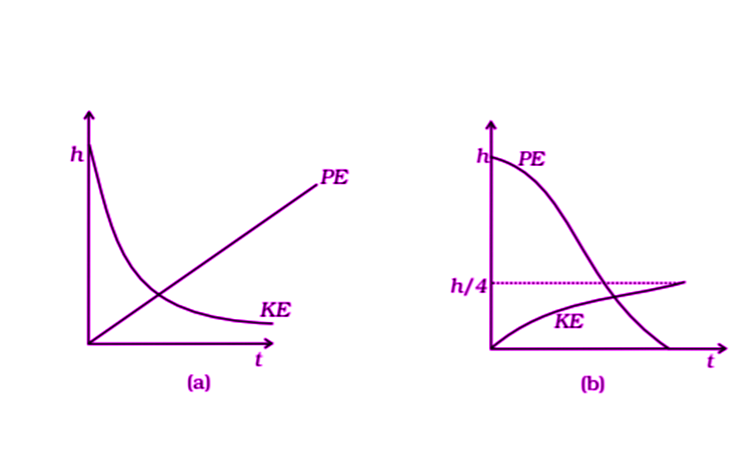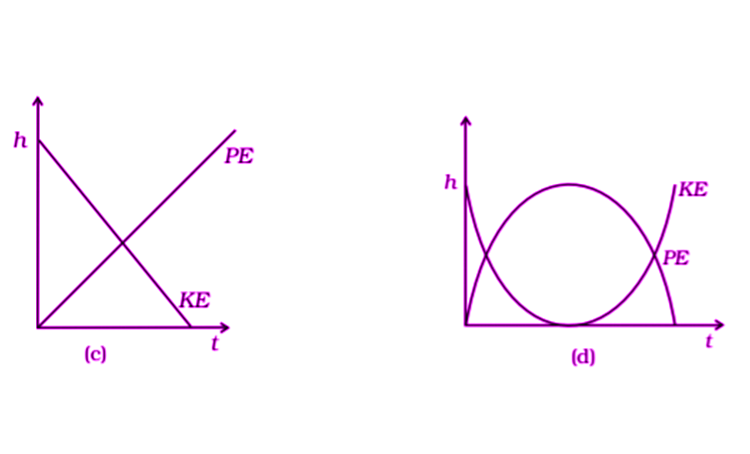b)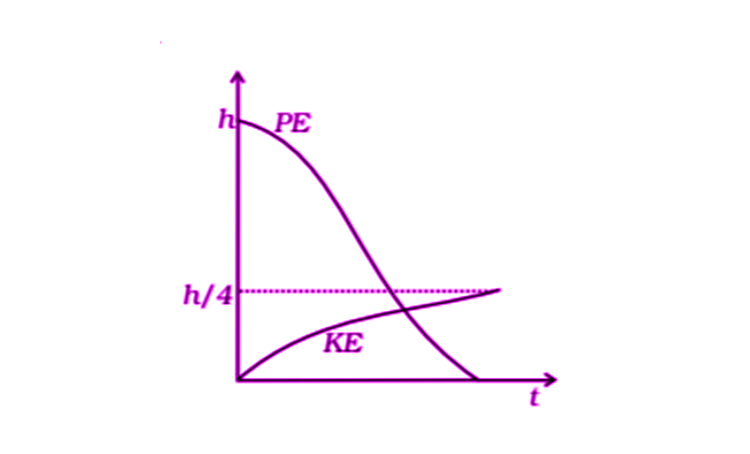6.16. In a shotput event an athlete throws the shotput of mass 10 kg with an initial speed of 1 m/s at 45o from height 1.5 m above ground. Assuming air resistance to be negligible and acceleration due to gravity to be 10 m/s2, the kinetic energy of the shotput when it just reaches the ground will be

a) 2.5 J

b) 5.0 J

c) 52.5 J

d) 155.0 J

6.17. Which of the diagrams in figure correctly shows the change in kinetic energy of an iron sphere falling freely in a lake having sufficient depth to impart it a terminal velocity?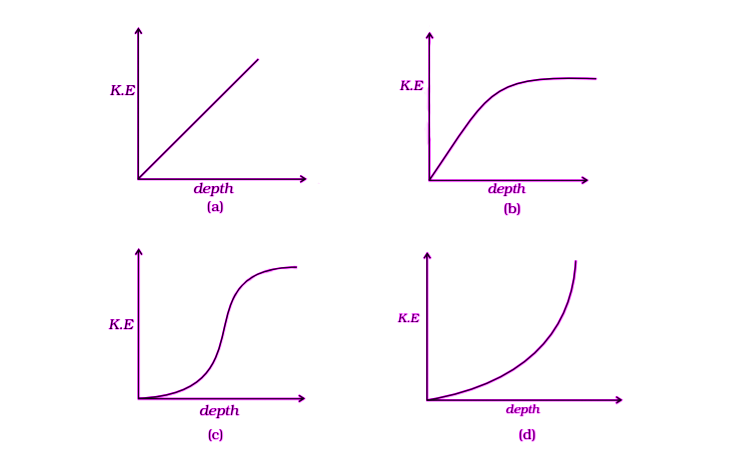b)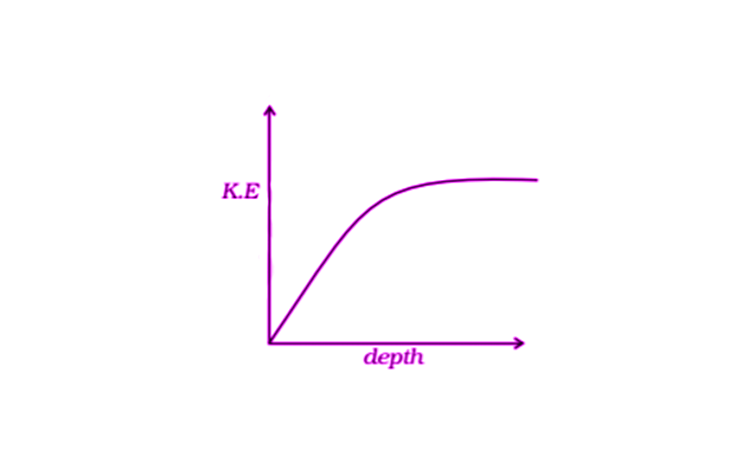6.18. A cricket ball of mass 150 g moving with a speed of 126 km/h hits at the middle of the bat, held firmly at its position by the batsman. The ball moves straight back to the bowler after hitting the bat. Assuming that collision between ball and bat is completely elastic and the two remain in contact for 0.001 sec, the force that the batsman had to apply to hold the bat firmly at its place would be

a) 10.5 N

b) 21 N

c) 1.05 × 104 N

d) 2.1 × 104 N

Answer: c) 1.05 × 104 N

### Multiple Choice Questions II

6.19. A man of mass m, standing at the bottom of the staircase, of height L, climbs it and stands at its top.

a) work done by all forces on man is zero

b) work done by all the force on man is zero

c) work done by the gravitational force on man is mgL

d) the reaction force from a step does not do work because the point of application of the force does not move while the force exists

b) work done by all the force on man is zero

d) the reaction force from a step does not do work because the point of application of the force does not move while the force exists

6.20. A bullet of mass m fired at 30o to the horizontal leaves the barrel of the gun with a velocity v. The bullet hits a soft target at a height h above the ground while it is moving downward and emerges out with half the kinetic energy it had before hitting the target. Which of the following statements are correct in respect of bullet after it emerges out of the target?

a) the velocity of the bullet will be reduced to half its initial value

b) the velocity of the bullet will be more than half of its earlier velocity

c) the bullet will continue to move along the same parabolic path

d) the bullet will move in a different parabolic path

e) the bullet will fall vertically downward after hitting the target

f) the internal energy of the particles of the target will increase

b) the velocity of the bullet will be more than half of its earlier velocity

d) the bullet will move in a different parabolic path

f) the internal energy of the particles of the target will increase

6.21. Two blocks M1 and M2 having equal mass are free to move on a horizontal frictionless surface. M2 is attached to a massless spring as shown in the figure. Initially, M2 is at rest and M1 is moving toward M2 with speed v and collides head-on with M2.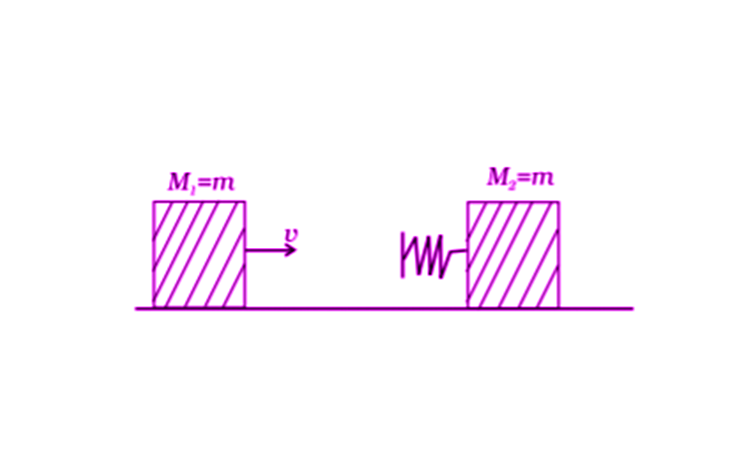a) while spring is fully compressed all the KE of M1 is stored as PE of spring

b) while spring is fully compressed the system momentum is not conserved, though final momentum is equal to the initial momentum

c) if spring is massless, the final state of the M1 is the state of rest

d) if the surface on which blocks are moving has friction, then a collision cannot be elastic

c) if spring is massless, the final state of the M1 is a state of rest

d) if the surface on which blocks are moving has friction, then a collision cannot be elastic

6.22. A rough inclined plane is placed on a cart moving with a constant velocity u on horizontal ground. A block of mass M rests on the incline. Is any work done by a force of friction between the block and incline? Is there then a dissipation of energy?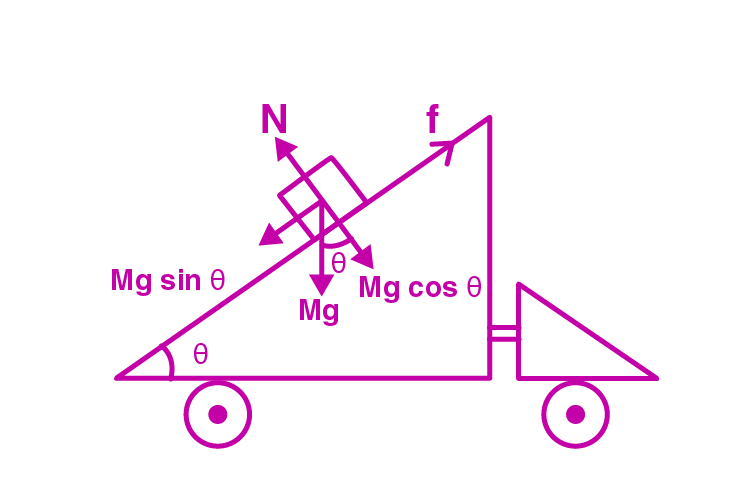From the above figure, it is clear that the block is inclined on the plane. There is no work done, as there is no displacement happening and no dissipation energy takes place.

6.23. Why is electrical power required at all when the elevator is descending? Why should there be a limit on the number of passengers in this case?

There is a limit on the number of passengers in case of an elevator descending as it is not a free fall and moves down with a uniform speed.

6.24. A body is being raised to a height h from the surface of the earth. What is the sign of work done by

a) applied force

b) gravitational force

a) The work done by the applied force is positive

b) The work done by the gravitational force is negative

6.25. Calculate the work done by a car against gravity in moving along a straight horizontal road. The mass of the car is 400 kg and the distance moved is 2m.

WD = Fs cos θ

WD = Fs cos 90o = 0

Therefore, the work done by the car against the gravity is zero.

6.26. A body falls towards earth in the air. Will its total mechanical energy be conserved during the fall? Justify.

The total mechanical energy of the free-falling body is no conserved as this energy is used against the force of friction from the air molecules.

6.27. A body is moved along a closed loop. Is the work done in moving the body necessarily zero? If not, state the condition under which work done over a closed path is always zero.

The work done by the moving body is zero when the conservative force is acting on the body during the motion. The work done by the moving body is non-zero when the non-conservative force is acting on the body.

6.28. In an elastic collision of two billiard balls, which of the following quantities remain conserved during the short time of collision of the balls

a) kinetic energy

b) total linear momentum

The kinetic energy and the total linear momentum of the billiard balls are conserved as there is no non-conservative force.

6.29. Calculate the power of a crane in watts, which lifts a mass of 100 kg to a height of 10 min 20 sec.

P = WD/time = Fs cos θ/t = mgh cos θ/t

h = 10 m

t = 20 sec

F = mg = 1000

Therefore, P = 500 Watts

6.30. The average work done by a human heart while it beats once is 0.5 J. Calculate the power used by heart if it beats 72 times in a minute.

P = WD/time

WD is one beat of heart = 0.5 J

WD in 72 beats = 36 J

P = WD/t = 0.6 W

6.31. Give an example of a situation in which an applied force does not result in a change in kinetic energy.

Work done during the circular motion there is no change in the kinetic energy.

6.32. Two bodies of unequal mass are moving in the same direction with equal kinetic energy. The two bodies are brought to rest by applying retarding force of the same magnitude. How would the distance moved by them before coming to rest compare?

KE1 = KE2

WD1 = WD2

F1s1 = F2s2

F1 = F2

s1 = s2

6.33. A bob of mass m suspended by a light string of length L is whirled into a vertical circle as shown in the figure. What will be the trajectory of the particle if the string is cut at

a) point B

b) point C

c) point X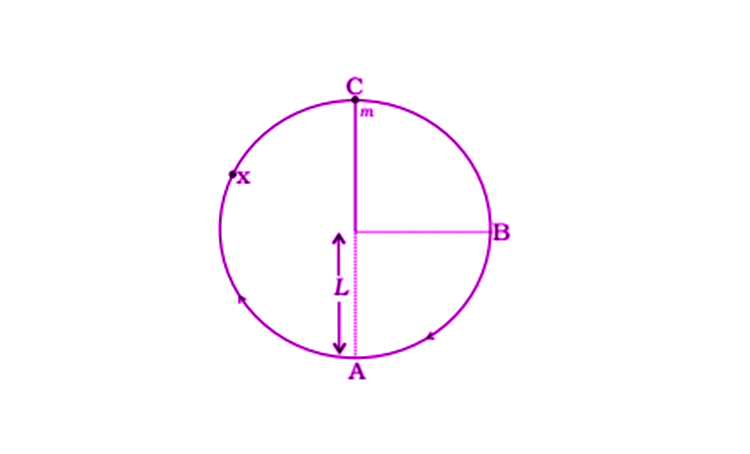a) The trajectory of the particle when the string is cut at point B is the tangential velocity will be vertically downward and the bob will move along the same path.

b) The trajectory of the particle when the string is cut at point C is the tangential velocity will be horizontal and will move in half parabolic path.

c) The trajectory of the particle when the string is cut at point X is when angle θ is formed and will move in the parabolic path till the bob reaches the highest point.

6.34. A graph of potential energy V(x) versus x is shown in the figure. A particle of energy E0 is executing motion in it. Draw graph of velocity and kinetic energy versus x for one complete cycle AFA.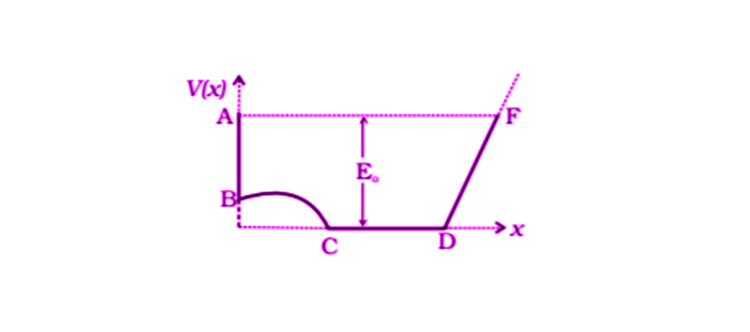From the given graph of KE versus x

 x KE Point 0 0 A 0 E1 B X1 E0 C X2 E0 D X3 0 F

From the below graph of velocity versus x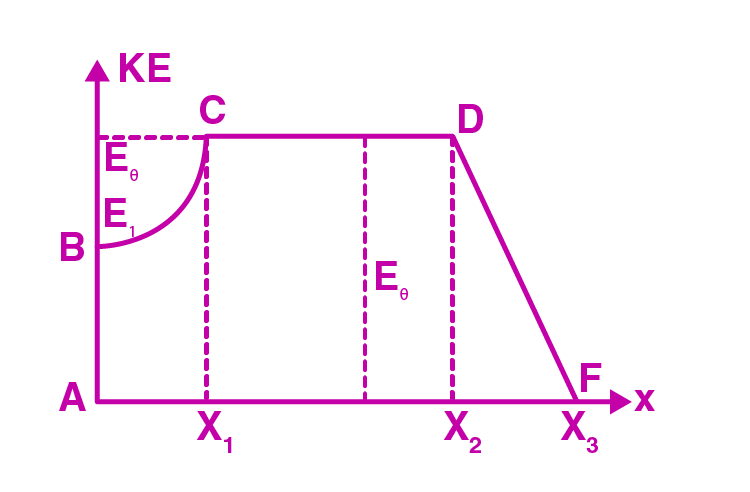Point V X A 0 0 B ±V1 0 C ±V0 X1 D ±V0 X2 E 0 X3

6.35. A ball of mass m, moving with speed 2v0, collides inelastically with an identical ball at rest. Show that

a) for a head-on collision, both the balls move forward

b) for a general collision, the angle between the two velocities of scattered balls is less than 90o.

a) Let v1 and v2 be the velocities of the two balls after the collision. According to the law of conservation of momentum,

mv0 = mv1 + mv2

v2 = v1 + 2ev0

e < 1

b)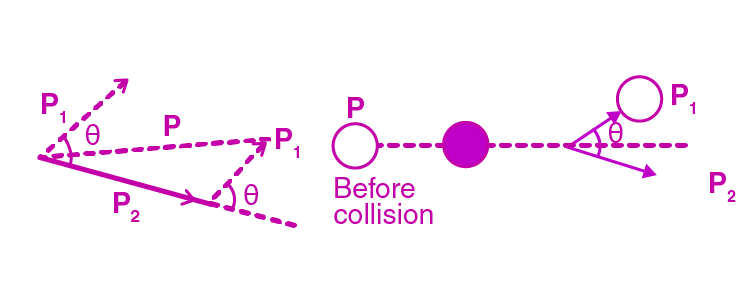According to the law of conservation of momentum,

p = p1 + p2

When the angle between the p1 and p2 is 90o, p > p1 + p2

6.36. Consider a one-dimensional motion of a particle with total energy E. There are four regions A, B, C, and D in which the relation between potential energy V, kinetic energy (K) and total energy is as given below:

Region A: V > E

Region B: V < E

Region C: K > E

Region D: V > K

State with reason in each case whether a particle can be found in the given region or not.

For region A, E = V + K and V > E which means that the KE is negative and this is not possible.

For region B, K = E – V and V < E which means that both the energies are greater than zero

For region C, V = E – K and K > E which means that PE is negative

For region D, K = E – V and V > K which means that PE is greater than KE

6.37. The bob A of a pendulum released from horizontal to the vertical hits another bob B of the same mass at rest on a table as shown in the figure. If the length of the pendulum is 1 m, calculate

a) the height to which bob A will rise after collision

b) the speed with which bob B starts moving.

Neglect the size of the bobs and assume the collision to be elastic.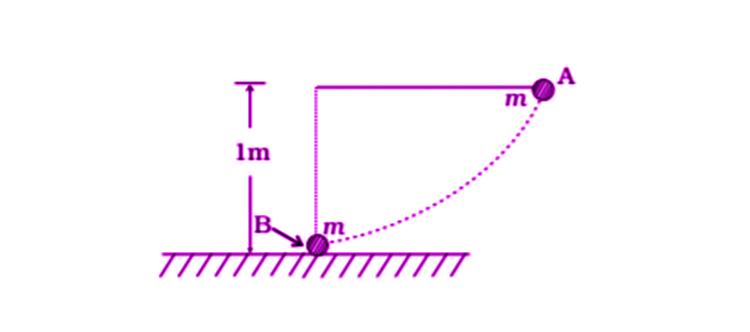a) There is not much rise in bob A after the collision as the PE of bob A gets converted in KE and the momentum is transferred to bob B.

b)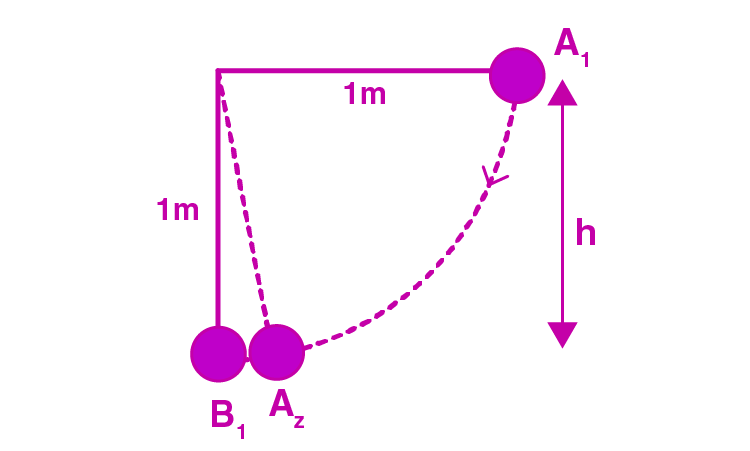The speed of bob B is found using the sum of KE of bob A and KE of bob B = 4.42 m/s

6.38. A raindrop of mass 1.00 g falling from a height of 1 km hits the ground with a speed of 50 m/s. Calculate

a) the loss of PE of the drop

b) the gain in KE of the drop

c) is the gain in KE equal to the loss of PE. If not, why?

a) PE at the highest point = 10 J

b) Gain in KE = 1/2 mv2 = 1.250 J

c) Gain in KE is not equal to the PE

6.39. Two pendulums with identical bobs and lengths are suspended from a common support such that in rest position the two bobs are in common. One of the bobs is released after being displaced by 10o so that it collides elastically head-on with the other bob.

a) describe the motion of two bobs

b) draw a graph showing variation in energy of either pendulum with time for 0 ≤ t ≤ 2T where T is the period of each pendulum

 Time t KEa PEa Ea KEb PEb Eb 0 0 0 0 0 E E T/4 E 0 E 0 0 0 2T/4 E 0 0 0 E E 3T/4 E 0 E 0 0 0 4T/4 = T 0 0 0 0 E E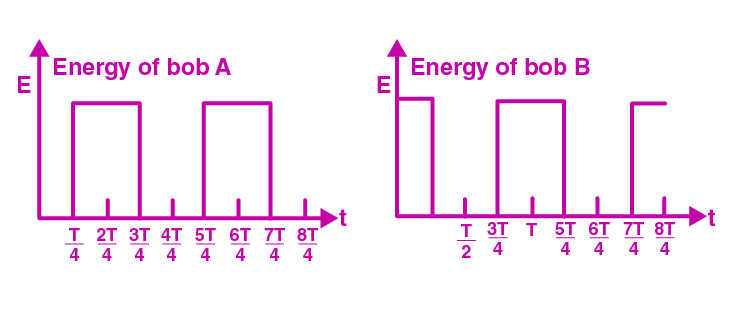6.40. Suppose the average mass of raindrops is 3.0 × 10-5 kg and their average terminal velocity 9 m/s. Calculate the energy transferred by rain to each square meter of the surface at a place which receives 100 cm of rain in a year.

Energy transferred by the rain to the surface of the earth = 1/2 mv2

The velocity of the rain = 9 m/s

Mass = (volume)(density) = 1000 kg

Energy transferred by 100 cm rainfall = 1/2 mv2 = 4.05×104 J

6.41. An engine is attached to a wagon through a shock absorber of length 1.5 m. The system with a total mass of 50,000 kg is moving with a speed of 36 km/h when the brakes are applied to bring it to rest. In the process of the system being brought to rest, the spring of the shock absorber gets compressed by 1.0 m. If 90% of the energy of the wagon is lost due to friction, calculate the spring constant.

KE = 1/2 mv2

m = 50000 kg

v = 10 m/s

KE = 2500000J

KE of spring = 10% of the KE wagon

K = 5 × 105 N/m

6.42. An adult weighing 600 N raises the centre of gravity of his body by 0.25 m while taking each step of 1 m length in jogging. If he jogs for 6 km, calculate the energy utilized by him in jogging, assuming that there is no energy loss due to friction of ground and air. Assuming that the body of the adult is capable of converting 10% of energy intake in the form of food, calculate the energy equivalents of food that would be required to compensate energy utilized for jogging.

The energy used up is given as = mgh

mg = 600 N

h = 0.25m

No.of steps in 6 km = 6000 steps

Energy used in 6000 m = (6000)(600)(0.25)J

Energy utilized in jogging = 9 × 104 J

6.43. On complete combustion, a litre of petrol gives off heat equivalent to 3 × 107 J. In a test drive a car weighing 1200 kg, including the mass of driver, runs 15 km per litre while moving with a uniform speed on a surface and air to be uniform, calculate the force of friction acting on the car during the test drive, if the efficiency of the car engine were 0.5.

Efficiency of the car engine = 0.5

Energy given by the car with 1 litre of petrol = 1.5 × 107

WD = 1.5 × 107

f = 103 N

6.44. A block of mass 1 kg is pushed up a surface inclined to horizontal at an angle of 30o by a force of 10 N parallel to the inclined surface. The coefficient of friction between the block and the incline is 0.1. If the block is pushed up by 10 m along the incline, calculate

a) work done against gravity

b) work done against the force of friction

c) increase in potential energy

d) increase in kinetic energy

e) work done by an applied force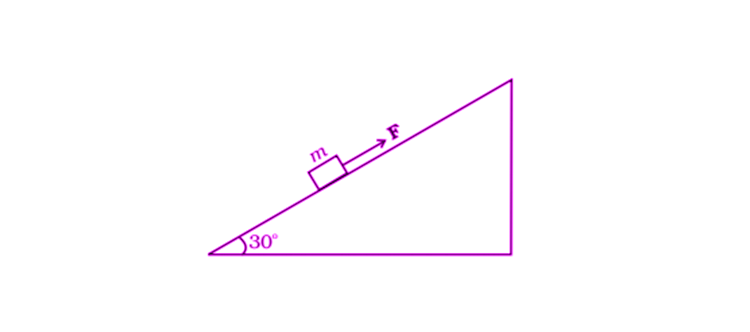a) Work done against gravity = mgh

h = 5 m

WD against gravity = 50 J

b) Work done against the force of friction is μfs = 5√3 J

c) Increase in PE = WD against the gravity = 50 J

d) Increase in KE is equal to the WD by the system = ∆K = WD = 41.340 J

e) WD by the applied force = Fs = 100J

6.45. A curved surface as shown in the figure. The portion BCD is free of friction. There are three spherical balls of identical radii and masses. Balls are released from one by one from A, which is at a slightly greater height than C. with the surface AB, ball 1 has large enough friction to cause rolling down without slipping; ball 2 has a small friction and ball 3 has a negligible friction.

a) for which balls is total mechanical energy conserved?

b) which ball can reach D?

c) for balls which do not reach D, which of the balls can reach back A?

a) For ball 1 the total mechanical energy is conserved

b) Ball 1 reaches D

c) Ball 3 reaches back A

6.46. A rocket accelerates straight up by ejecting gas downwards. In a short time interval ∆t, it ejects a gas of mass ∆m at a relative speed u. Calculate KE of the entire system at t + ∆t and t and show that the device that ejects gas does work = (1/2) ∆m u2 in this time interval.

M is the mass of the rocket at any time t

v is the velocity of the rocket

∆m is the mass of the gas that is ejected during the time interval ∆t

Therefore, K = 1/2 u2∆m

6.47. Two identical steel cubes collide head-on face to face with a speed of 10 cm/s each. Find the maximum compression of each. Young’s modulus for steel = Y = 2 × 1011 N/m2.

Y = stress/strain

Y = FL/A∆L

WD = F∆L

KE = 5 × 10-4 J

WD = KE

∆L = 5 × 10-7 m

6.48. A balloon filled with helium rises against gravity increasing its potential energy. The speed of the balloon also increases as it rises. How do you reconcile this with the law of conservation of mechanical energy? You can neglect the viscous drag of air and assume that the density of air is constant.

When the dragging viscous force of the air on the balloon is neglected, then the net buoyant force = vpg

Where v is the volume of air displaced

p is the net density upward

After calculation, it can be said that as the balloon goes up, the sum of kinetic energy and the potential energy of the balloon will be equal to change in potential energy in the air.

#### Important Topics of Chapter 6 Work, Energy and Power

 Notions of work and kinetic energy: The work-energy theorem Work Kinetic energy Work done by a variable force The work-energy theorem for a variable force The concept of potential energy The conservation of mechanical energy The potential energy of a spring Forms of energy: the law of conservation of energy Power Collisions

### Why Opt for BYJU’S?

BYJU’S presents you with the best study materials, notes, books, previous years’ question papers, sample papers, numerical problems, videos and animations, which will help you prepare for the Class 11 examination and graduate entrance examinations. At BYJU’S, you will get NCERT solutions for all chapters of all the subjects of Class 11. For an effective and interactive learning experience, download BYJU’S – The Learning App.